# Autoencoders, latent space and the curse of high dimensionality – II – a view on fragments and filaments of the latent space for CelebA images

I continue with experiments regarding the structure which an Autoencoder [AE] builds in its latent space. In the last post of this series

Autoencoders, latent space and the curse of high dimensionality – I

we have trained an AE with images of the CelebA dataset. The Encoder and the Decoder of the AE consist of a series of convolutional layers. Such layers have the ability to extract characteristic patterns out of input (image) data and save related information in their so called feature maps. CelebA images show human heads against varying backgrounds. The AE was obviously able to learn the typical features of human faces, hair-styling, background etc. After a sufficient number of training epochs the AE’s Encoder produces “z-points” (vectors) in the latent space. The latent space is a vector space which has a relatively low number of dimension compared with the number of image pixels. The Decoder of the AE was able to reconstruct images from such z-points which resembled the original closely and with good quality.

We saw, however, that the latent space (or “z-space”) lacks an important property:

The latent space of an Autoencoder does not appear to be densely and uniformly populated by the z-points of the training data.

We saw that his makes the latent space of an Autoencoder almost unusable for creative and generative purposes. The z-points which gave us good reconstructions in the sense of recognizable human faces appeared to be arranged and positioned in a very special way within the latent space. Below I call a CelebA related z-point for which the Decoder produces a reconstruction image with a clearly visible face a “meaningful z-point“.

We could not reconstruct “meaningful” images from randomly chosen z-points in the latent space of an Autoencoder trained on CelebA data. Randomly in the sense of random positions. The Decoder could not re-construct images with recognizable human heads and faces from almost any randomly positioned z-point. We got the impression that many more non-meaningful z-points exist in latent space than meaningful z-points.

We would expect such a behavior if the z-points for our CelebA training samples were arranged in tiny fragments or thin (and curved) filaments inside the multidimensional latent space. Filaments could have the structure of

• multi-dimensional manifolds with almost no extensions in some dimensions
• or almost one-dimensional string-like manifolds.

The latter would basically be described by a (wiggled) thin curve in the latent space. Its extensions in other dimensions would be small.

It was therefore reasonable to assume that meaningful z-points are surrounded by areas from which no reasonable interpretable image with a clear human face can be (re-) constructed. Paths from a “meaningful” z-point would only in a very few distinct directions lead to another meaningful point. As it would be the case if you had to follow a path on a thin curved manifold in a multidimensional vector space.

So, we had some good reasons to speculate that meaningful data points in the latent space may be organized in a fragmented way or that they lie within thin and curved filaments. I gave my readers a link to a scientific study which supported this view. But without detailed data or some visual representations the experiments in my last post only provided indirect indications of such a complex z-point distribution. And if there were filaments we got no clue whether these were one- or multidimensional.

Do we have a chance to get a more direct evidence about a fragmented or filamental population of the latent space? Yes, I think so. And this is the topic of this post.

However, the analysis is a bit complicated as we have to deal with a multidimensional space. In our case the number of dimensions of the latent space is z_dim = 256. No chance to plot any clusters or filaments directly! However, some other methods will help to reduce the dimensionality of the problem and still get some valid representations of the data point correlations. In the end we will have a very strong evidence for the existence of filaments in the AE’s z-space.

# Methods to work with data distributions in many dimensions

Below I will use several methods to investigate the z-point distribution in the multidimensional latent space:

• An analysis of the variation of the z-point number-density along coordinate axes and vs. radius values.
• An application of t-SNE projections from the standard multidimensional coordinate system onto a 2-dimensional plane.
• PCA analysis and subsequent t-SNE projections of the PCA-transformed z-point distribution and its most important PCA components down to a 2-dim plane. Note that such an approach corresponds to a sequence of projections:
1) Linear projections onto PCA rotated coordinates.
2) A non-linear SNE-projection which scales and represents data point correlations on different scales on a 2-dim plane.
• A direct view on the data distribution projected onto flat planes formed by two selected coordinate axes in the PCA-coordinate system. This will directly reveal whether the data (despite projection effects exhibit filaments and voids on some (small ?) scales.
• A direct view on the data distribution projected onto a flat plane formed by two coordinate axes of the original latent space.

The results of all methods combined strongly support the claim that the latent space is neither populated densely nor uniformly on (small) scales. Instead data points are distributed along certain filamental structures around voids.

# Layer structure of the Autoencoder

Below you find the layer structure of the AE’s Encoder. It got four Conv2D layers. The Decoder has a corresponding reverse structure consisting of Conv2DTranspose layers. The full AE model was constructed with Keras. It was trained on CelebA for 24 epochs with a small step size. The original CelebA images were reduced to a size of 96×96 pixels.

# Number density of z-points vs. coordinate values

Each z-point can be described by a vector, whose components are given by projections onto the 256 coordinate axes. We assume orthogonal axes. Let us first look at the variation of the z-point number density vs. reasonable values for each of the 256 vector-components.

Below I have plotted the number density of z-points vs. coordinate values along all 256 coordinate axes. Each curve shows the variation along one of the 256 axes. The data sampling was done on intervals with a width of 0.25: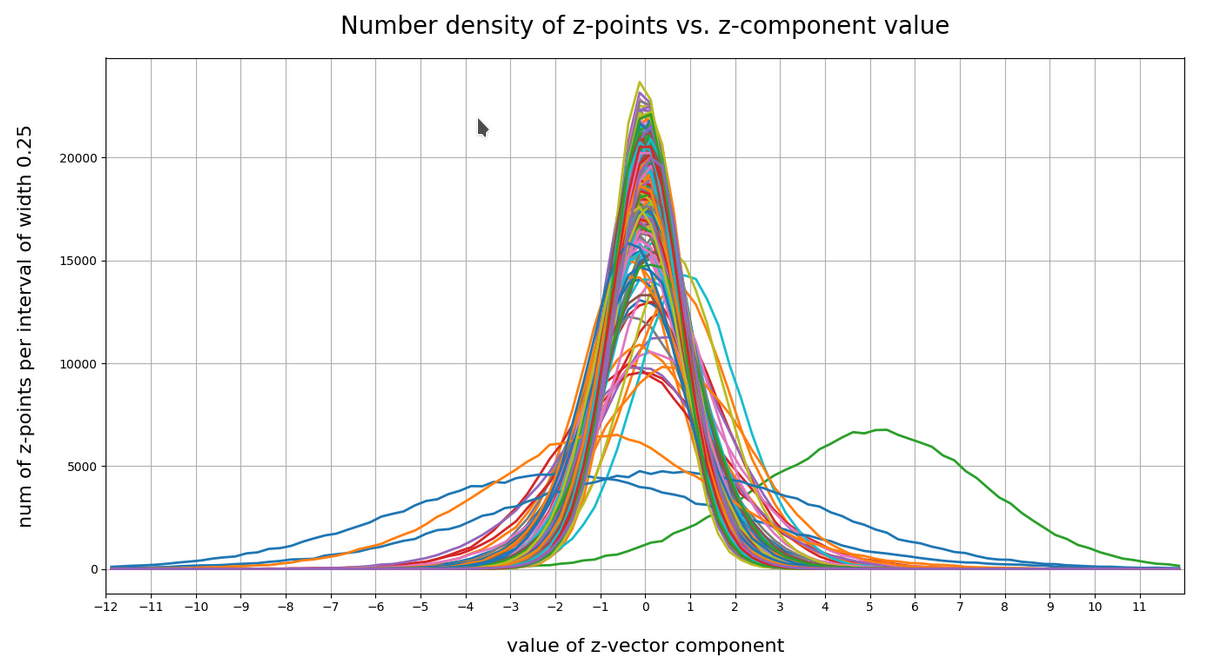Most curves look like typical Gaussians with a peak at the coordinate value 0.0 with a half-width of around 2.

You see, however, that there are some coordinates which dominate the spatial distribution in the latent vector-space. For the following components the number density distribution is relatively broad and peaks at a center different from the origin of the z-space. To pick a few of these coordinate axes:

``` 52, center:  5.0,  width: 8
61; center;  1.0,  width: 3
73; center:  0.0,  width: 5.5
83; center: -0.5,  width: 5
94; center:  0.0,  width: 4
116; center:  0.0,  width: 4
119; center:  1.0,  width: 3
130; center: -2.0,  width: 9
171; center:  0.7,  width: 5
188; center:  0.75, width: 2.75
200; center:  0.5,  width: 11
221; center: -1.0,  width: 8
```

The first number is just an index of the vector component and the related coordinate axis. The next plot shows the number density along some these specific coordinate axes:

What have we learned?
For most coordinate axes of the latent space the number density of the z-points peaks at 0.0. We see an approximate Gaussian form of the number density distribution. There are around 5 coordinate directions where the distribution has a peak significantly off the origin (52, 130, 171, 200, 221). Along the corresponding axes the distribution of z-points obviously has an elongated form.

If there were only one such special vector component then we would speak of an elongated, ellipsoidal and almost cigar like distribution with the thickest area at some position along the specific coordinate axis. For a combination of more axes with elongated distributions, each with with a center off the origin, we get instead diagonally oriented multidimensional and elongated shapes.

These findings show again that large regions of the latent space of an AE remain empty. To get an idea just imagine a three dimensional space with all data in x-direction culminating at a coordinate value of 5 with a half-width of lets say 8. In the other directions y and z we have our Gaussian distributions with a total half-width of 1 around the mean value 0. What do we get? A cigar like shape confined around the x-axis and stretching from -3 < x < 13. And the rest of the space: More or less empty. We have obviously found something similar at different angular directions of our multidimensional latent space. As the number of special coordinate directions is limited these findings tell us that a PCA analysis could be helpful. But let us first have a look at the variation of number density with the radius value of the z-points.

# Number density of z-points vs. radius

We define a radius via an Euclidean L2 norm for our 256-dimensional latent space. Afterward we can reduce the visualization of the z-point distribution to a one dimensional problem. We can just plot the variation of the number density of z-points vs. the radius of the z-points.

In the first plot below the sampling of data was done on intervals of 0.5 .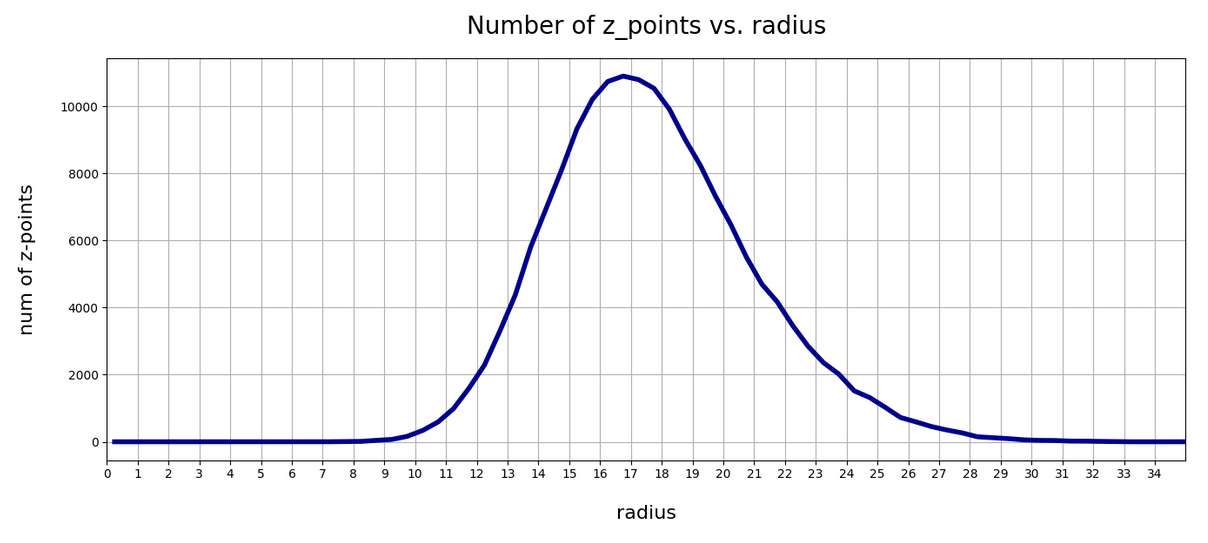The curve does not remain that smooth on smaller sampling intervals. See e.g. for intervals of width 0.05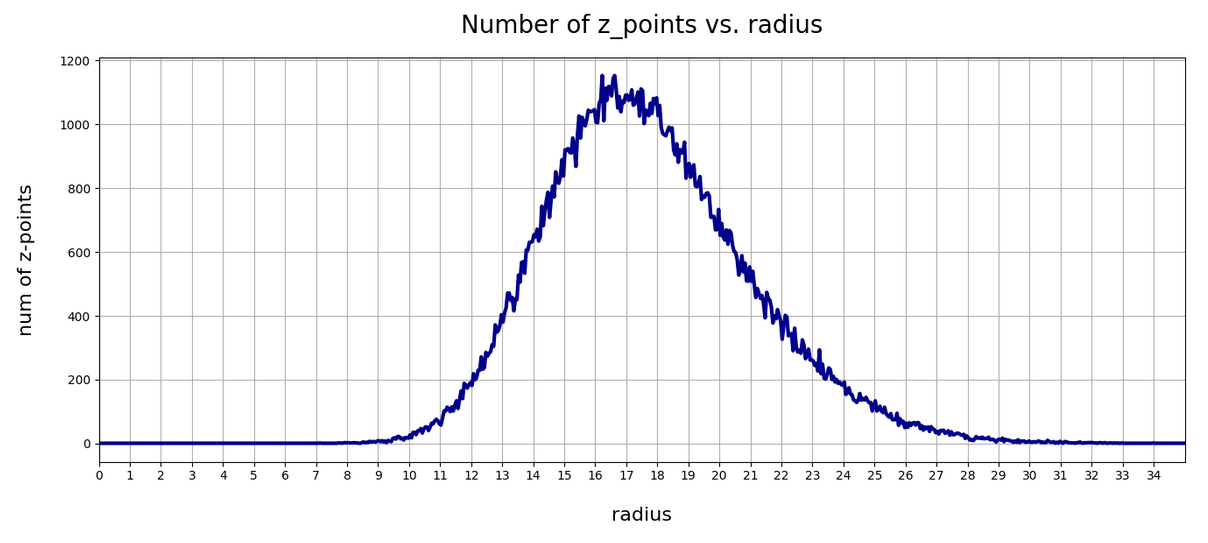Still, we find a pronounced peak at a radius of R=16.5. But do not get misguided: 16 appears to be a big value. But this is mainly due to the high number of dimensions!

How does the peak in the close vicinity of R=16 fit to the above number density data along the coordinate axes? Answer: Very well. If you assume a z-point vector with an average value of 1 per coordinate direction we actually get a radius of exactly R=16!

But what about Gaussian distributions along the coordinate axes? Then we have to look at resulting expectation values. Let us assume that we fill a vector of dimension 256 with numbers for each component picked statistically from a normal distribution with a width of 1. And let us repeat this process many times. Then what will the expectation value for each component be?

A coordinate value contributes with its square to the radius. The math, therefore, requires an evaluation of the integral integral[(x**2)*gaussian(x)] per coordinate. This integral gives us an expectation value for the contribution of each coordinate to the total vector length (on average). The integral indeed has a resulting value of 1.0. From this it follows that the expectation value for the distance according to an Euclidean L2-metric would be avg_radius = sqrt(256) = 16. Nice, isn’t it?

However, due to the fact that not all Gaussians along the coordinate axes peak at zero, we get, of course, some deviations and the flank of the number distribution on the side of larger radius values becomes relatively broad.

What do we learn from this? Regions very close to the origin of the z-space are not densely populated. And above a radius value of 32, we do not find z-points either.

# t-SNE correlation analysis and projections onto a 2-dimensional plane

To get an impression of possible clustering effects in the latent space let us apply a t-SNE analysis. A non-standard parameter set for the sklearn-variant of t-SNE was chosen for the first analysis

```tsne2 = TSNE(n_components, early_exaggeration=16, perplexity=10, n_iter=1000)
```

The first plot shows the result for 20,000 randomly selected z-points corresponding to CelebA images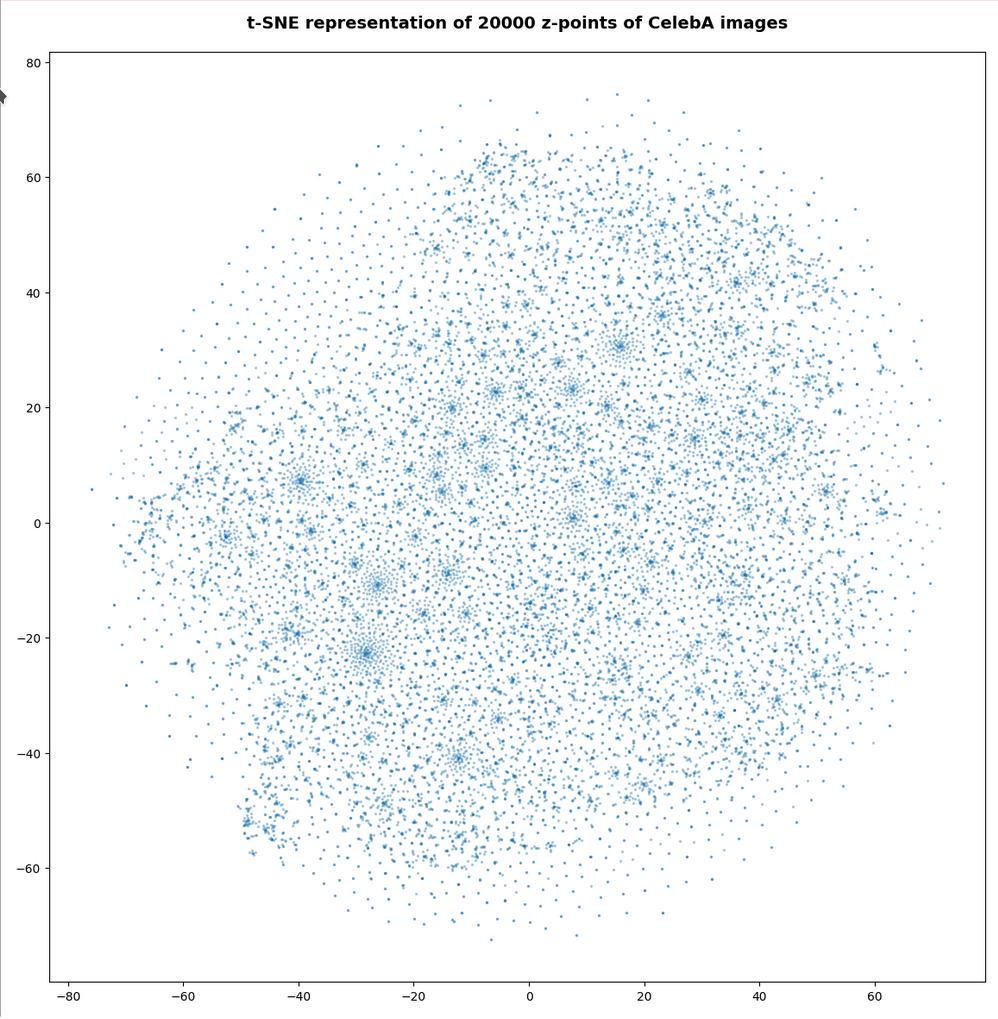Also this plot indicates that the latent space is not populated with uniform density in all regions. Instead we see some fragmentation and clustering. But note that this might happened on different length scales. t-SNE arranges its projections such that correlations on different scales get clearly indicated. So the distances in this plot must not be confused with the real spatial distances in the original latent space. The axes of the t-SNE plot do not reflect any axes of the latent space and the plotted distribution is not the real data point distribution after a linear and orthogonal projection onto a plane. t-SNE works non-linearly.

However, the impression of clustering remains for a growing numbers of z-points. In contrast to the first plot the next plots for 80,000 and 165,000 z-points were calculated with standard t-SNE parameters.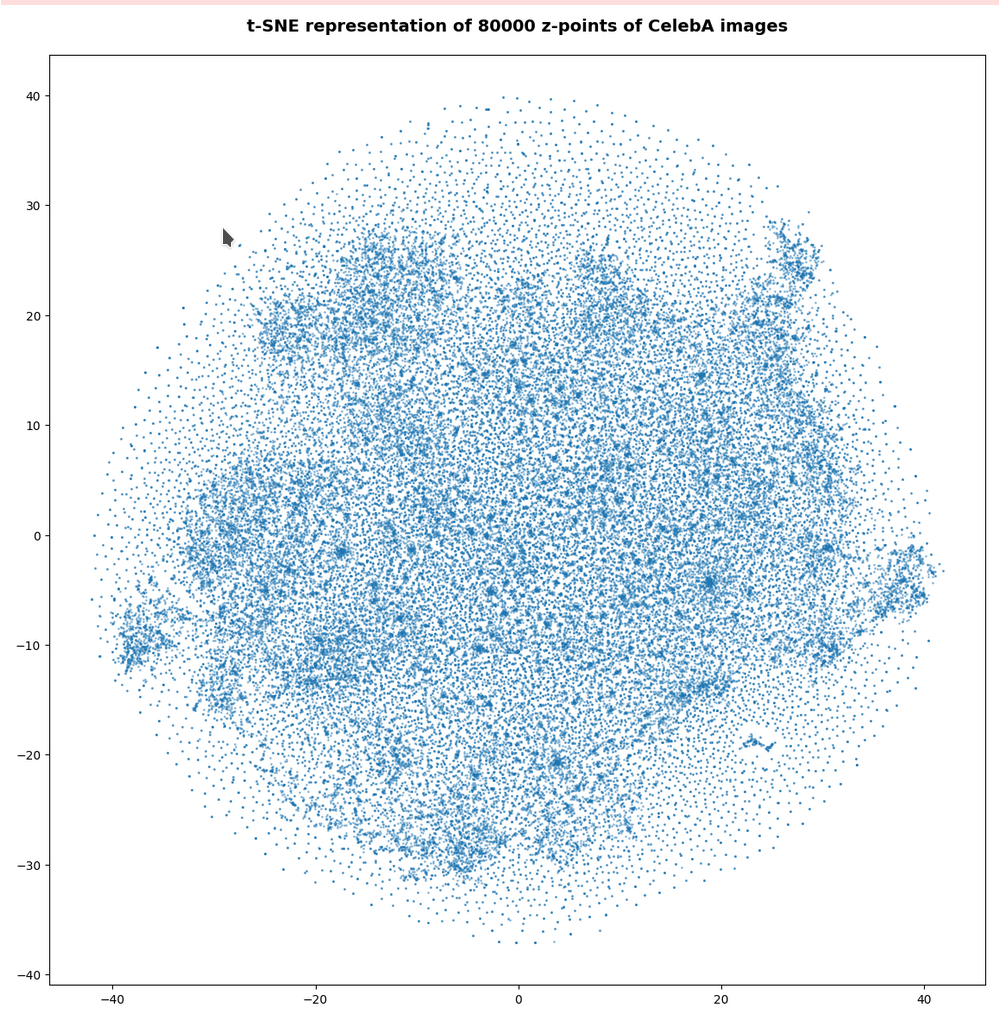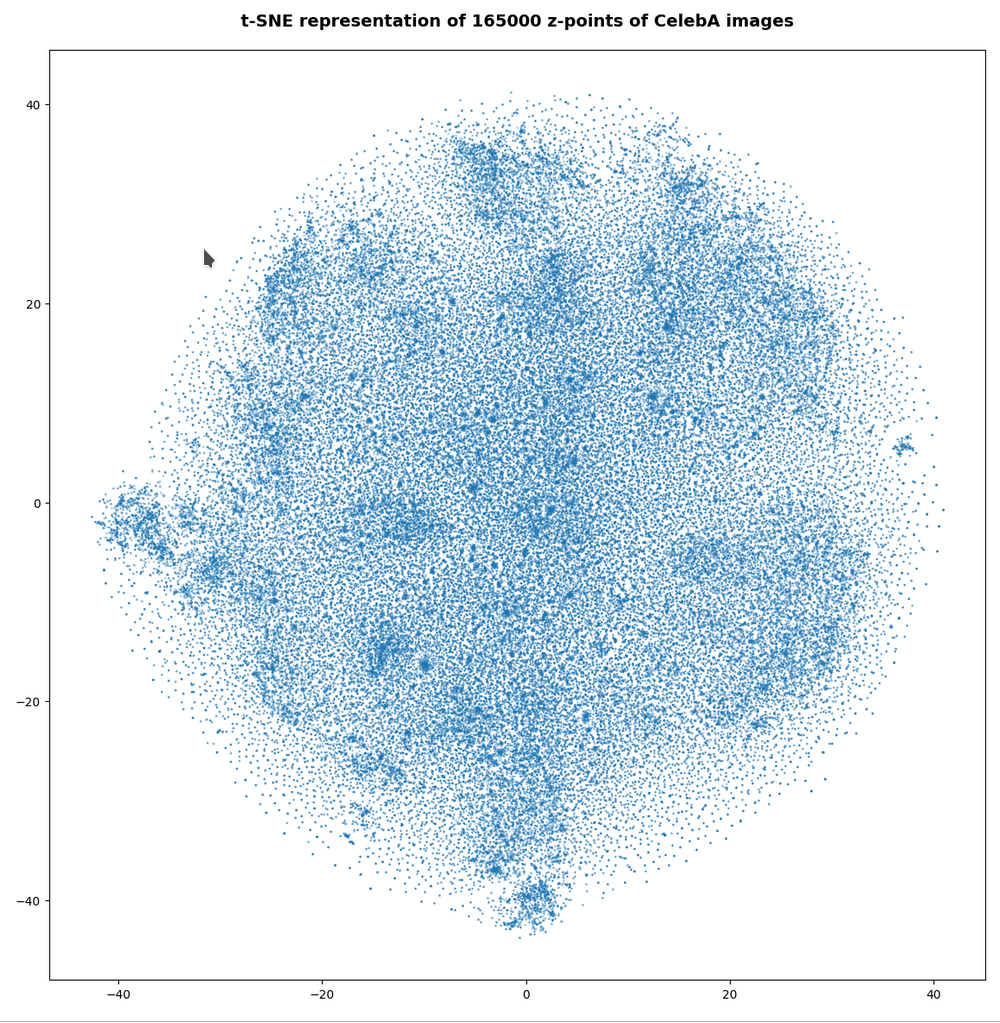We still see gaps everywhere between locally dense centers. At the center the size of the plotted points leads to overlapping. If one could zoom into some of the centers then gaps would again appear on smaller scales (see more plots below).

# PCA analysis and t-SNE-plots of the z-point distribution in the (rotated) PCA coordinate system

The z-point distribution can be analyzed by a PCA algorithm. There is one dominant component and the importance smooths out to an almost constant value after the first 10 components.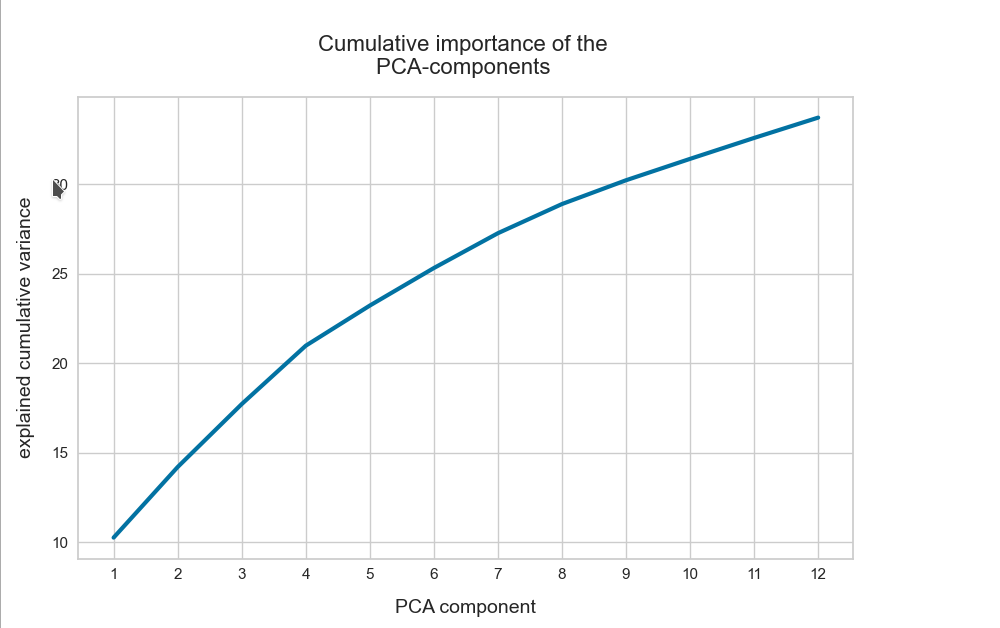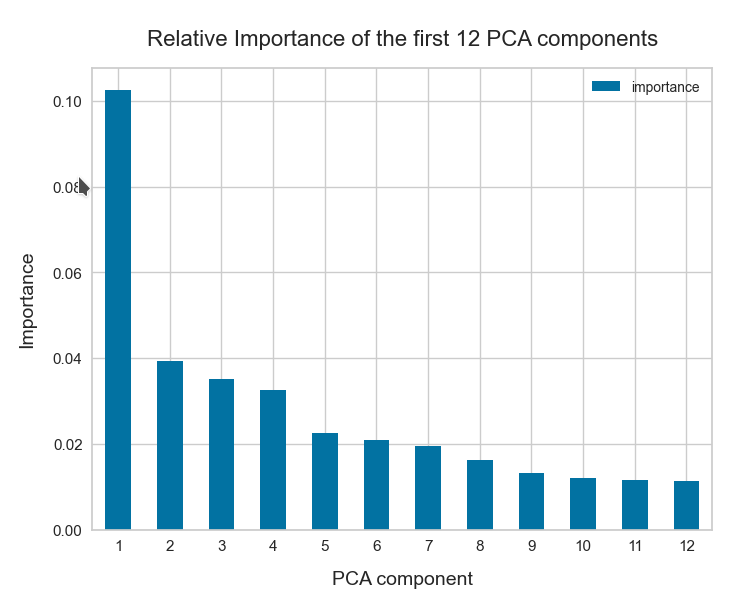This is consistent with the above findings. Most of the coordinates show rather similar Gaussian distributions and thus contribute in almost the same manner.

The PCA-analysis transforms our data to a rotated coordinate system with a its origin at a position such that the transformed z-point distribution gets centered around this new origin. The orthogonal axes of the new PCA-coordinates system show into the direction of the main components.

When the projection of all points onto planes formed by two selected PCA axes do not show a uniform distribution but a fragmented one, then we can safely assume that there really is some fragmentation going on.

## t-SNE after PCA

Below you see t-SNE-plots for a growing number of leading PCA components up to 4. The filamental structure gets a bit smeared out, but it does not really disappear. Especially the elongated empty regions (voids) remain clearly visible.

t-SNE after PCA for the first 2 main components – 80,000 randomly selected z-points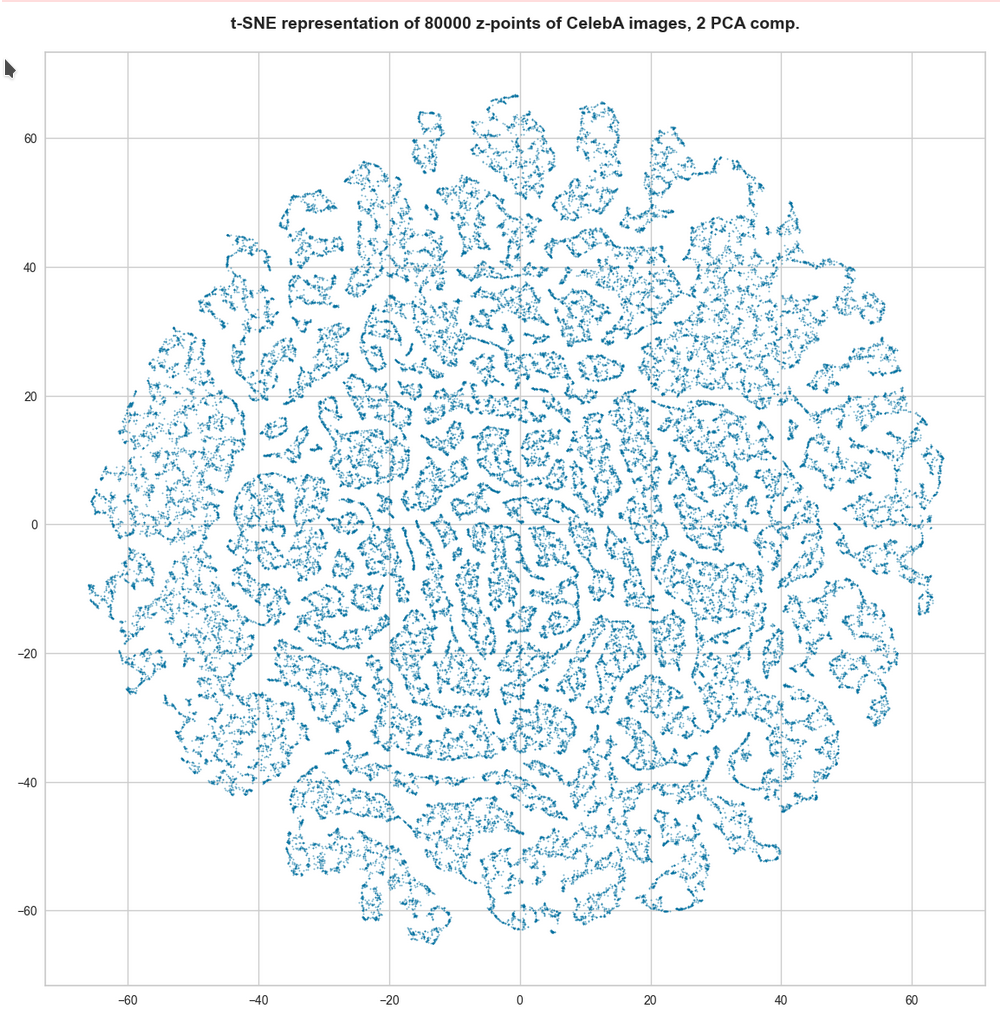t-SNE after PCA for the first 2 main components – 165,000 randomly selected z-points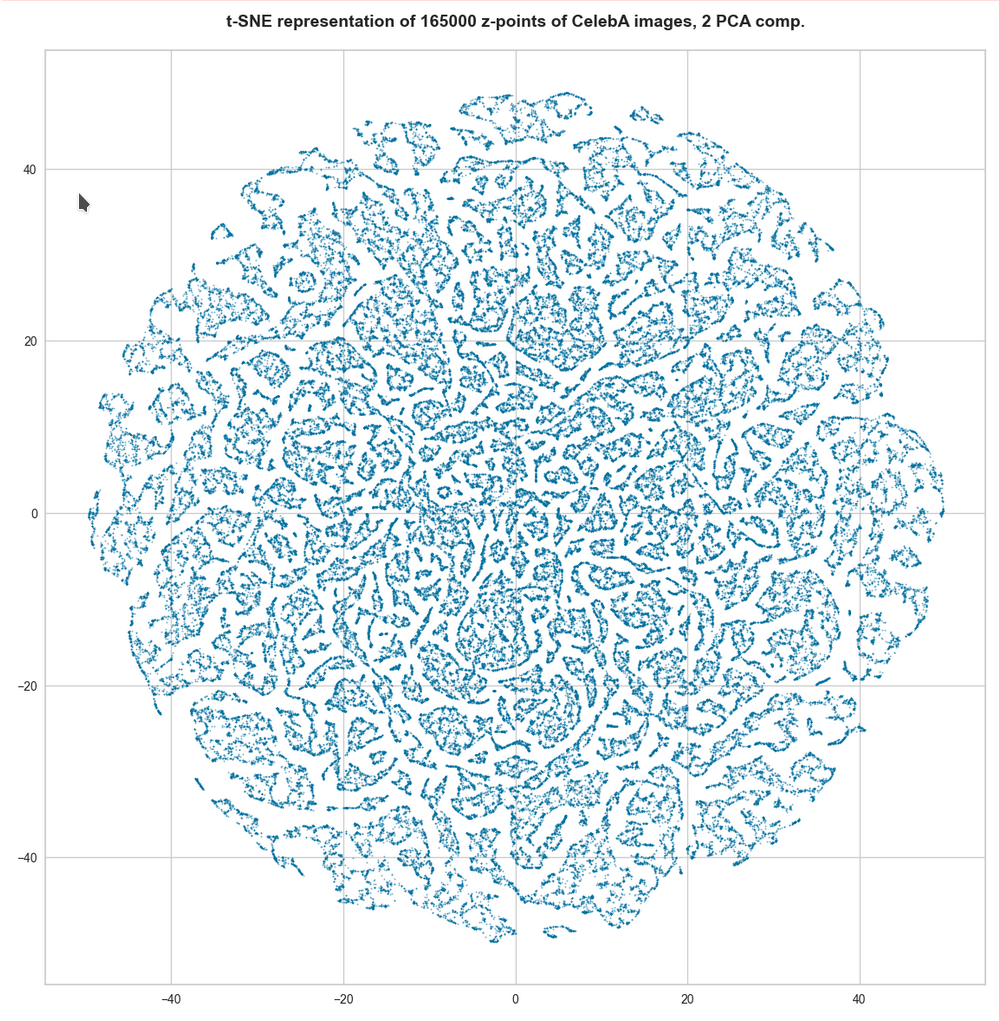t-SNE after PCA for the first 4 main PCA components – 165,000 randomly selected z-points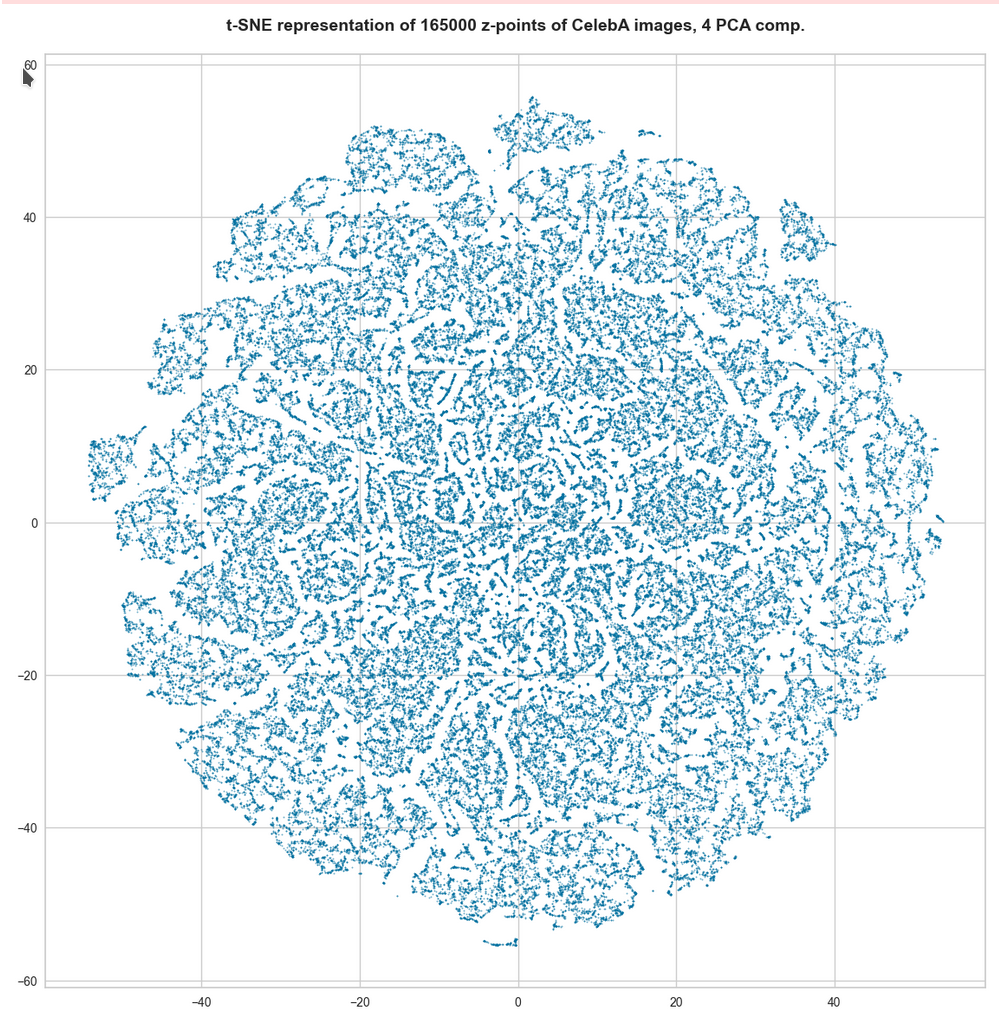For 10 components t-SNE gets a presentation problem and the plots get closer to what we saw when we directly operated on the latent space.But still the 10-dim space does not appear to be uniformly populated. Despite an expected smear out effect due to the non-linear projection the empty ares seem to be at least as many and as extended as the populated areas.

# Direct view on the z-point distribution after PCA in the rotated and centered PCA coordinate system

t-SNE blows correlations up to make them clearly visible. Therefore, we should also answer the following question:

On what scales does the fragmentation really happen ?

For this purpose we can make a scatter plot of the projection of the z-points onto a plane formed by the leading two primary component axes. Let us start with an overview and relatively large limiting values along the two (PCA) axes: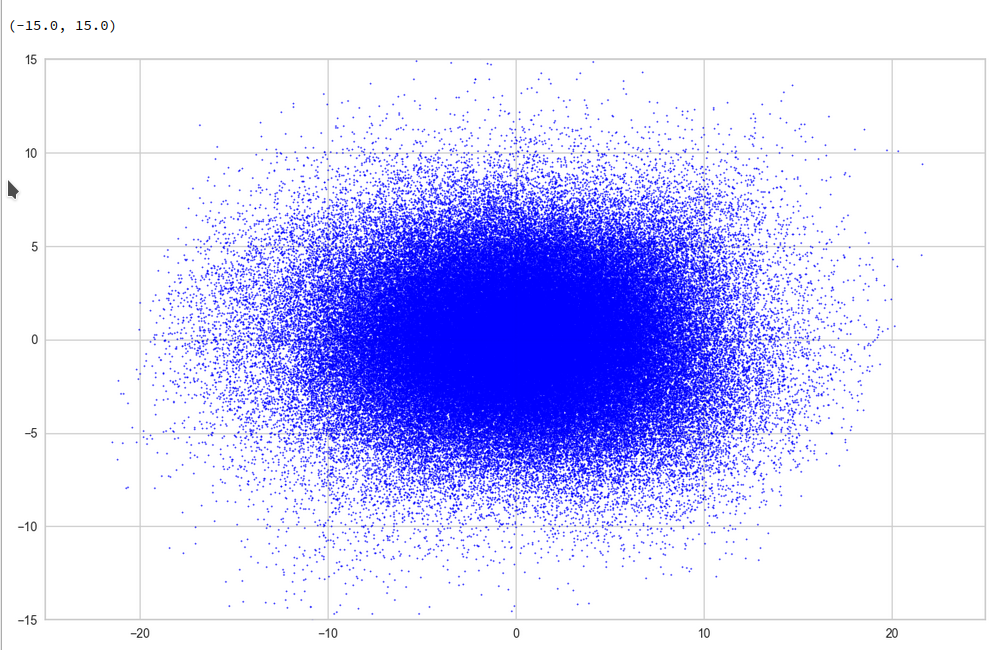Yeah, a PCA transformation obviously has centered the distribution. But now the latent space appears to be filled densely and uniformly around the new origin. Why?

Well, this is only a matter of the visualized length scales. Let us zoom in to a square of side-length 5 at the center: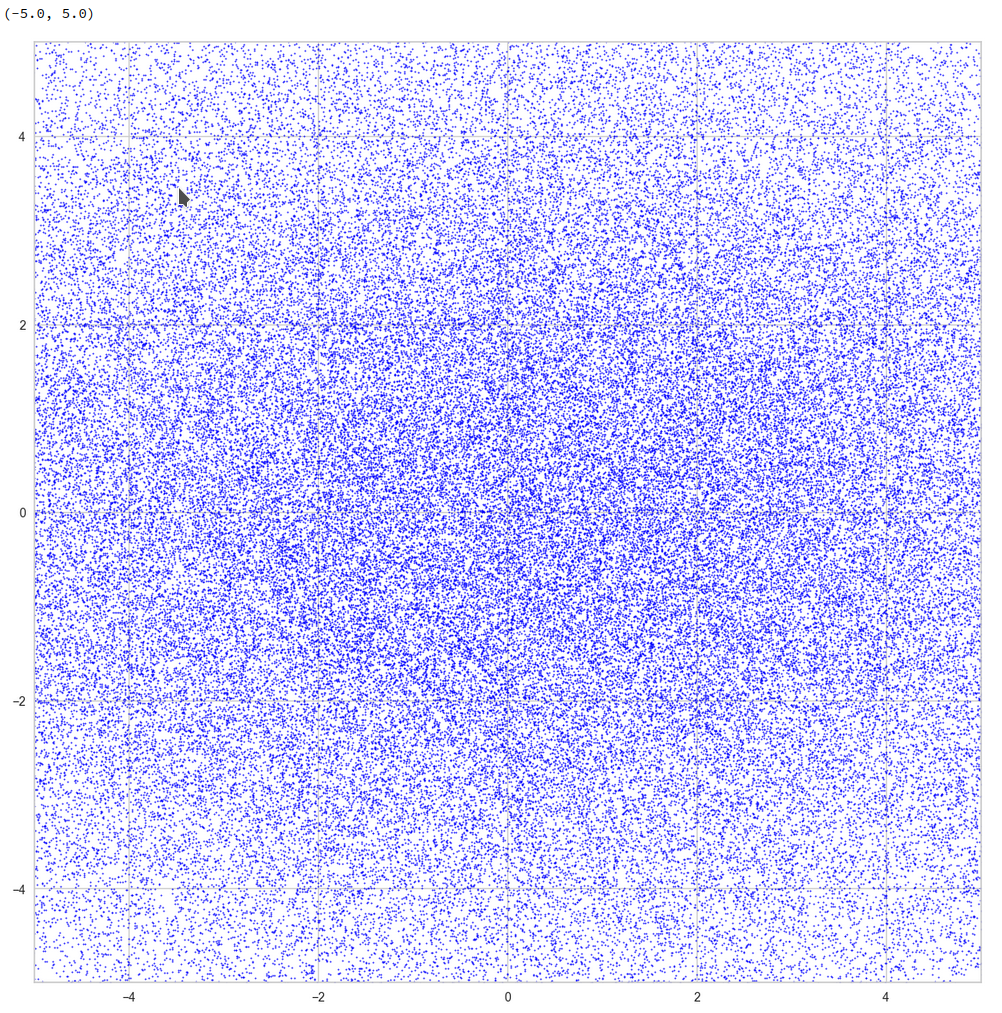Well, not so densely populated as we thought.

And yet a further zoom to smaller length scales: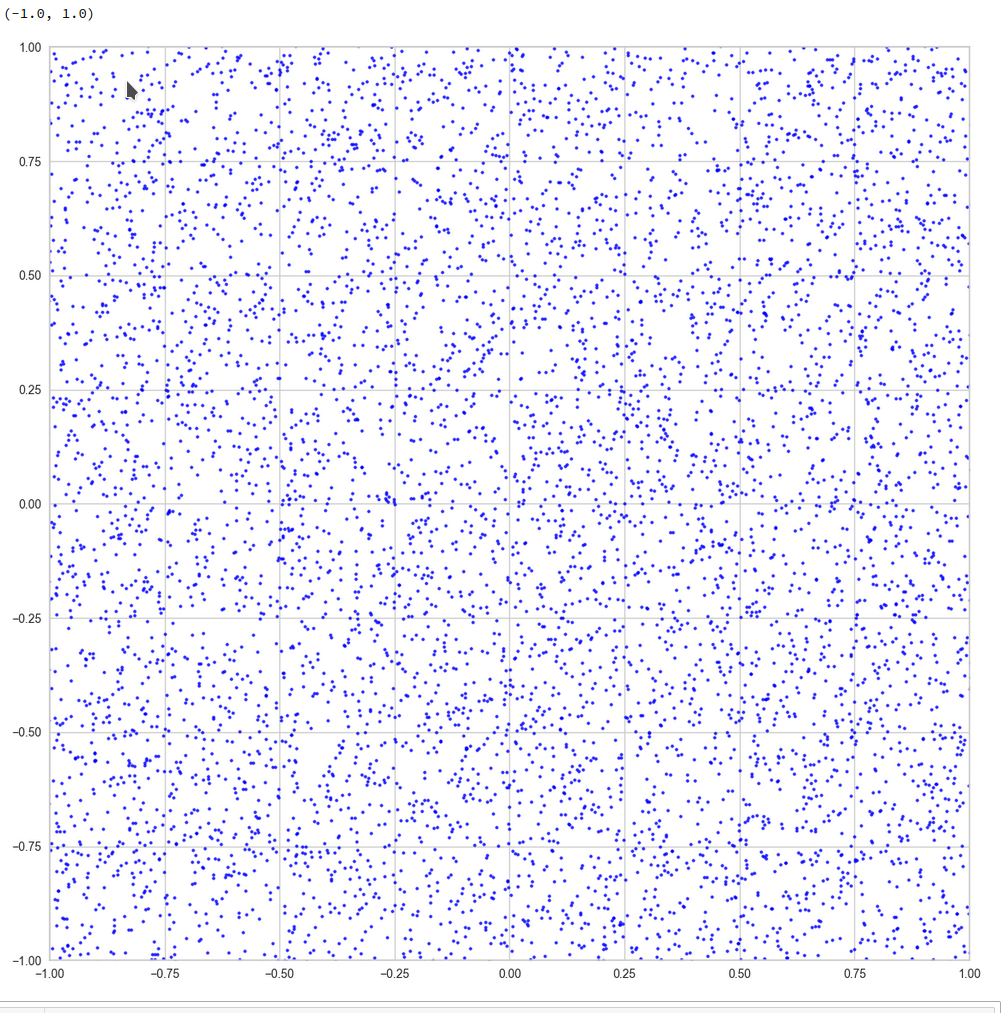And eventually a really small square around the origin of the PCA coordinate system:

z-point distribution at the center of a two-dim plane formed by the coordinate axes of the first 2 primary components
The chosen qsquare has its corners at (-0.25, -0.25), (-0.25, 0.25), (0.25, -0.25), (0.25, 0.25).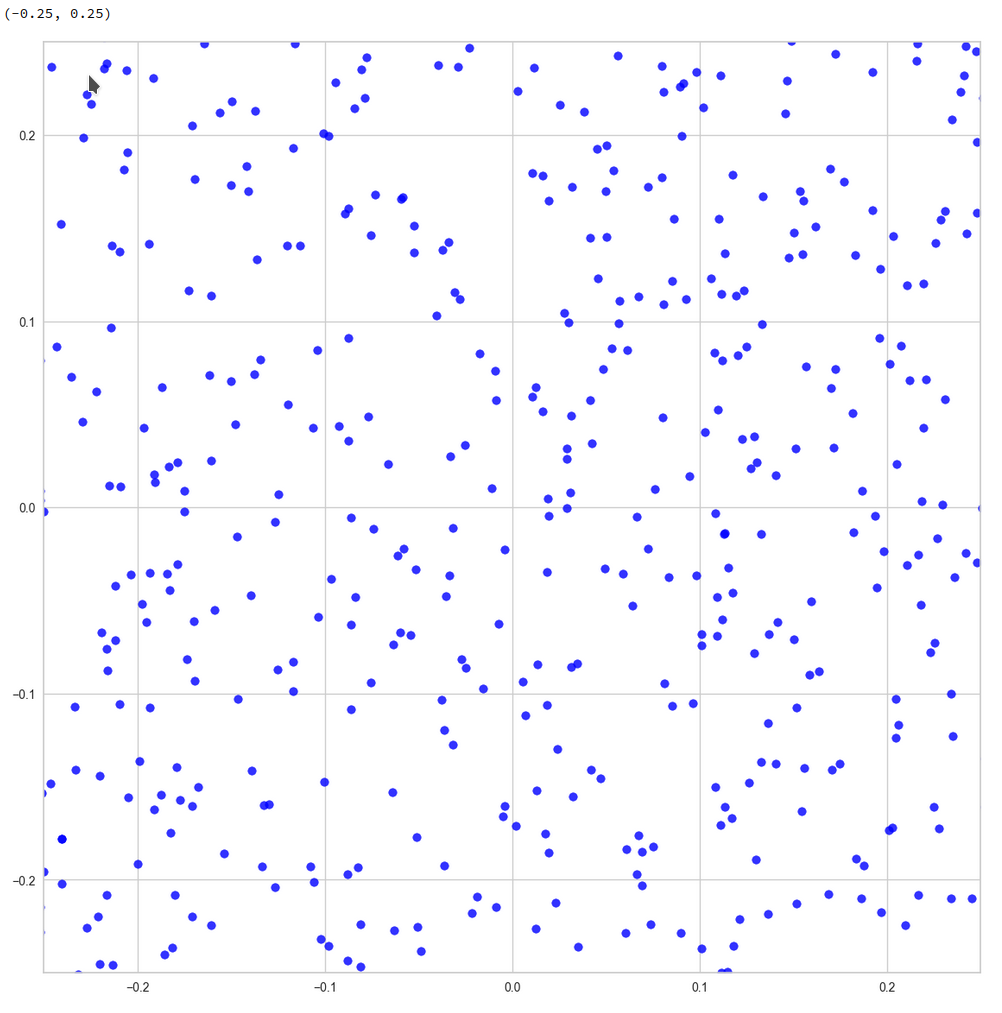Obviously, not a dense and neither a uniform distribution! After a PCA transformation we see the still see how thinly the latent space is populated and that the “meaningful” z-points from the CelebA data lie along curved and narrow lines or curves with some point-like intersections. Between such lines we see extended voids.

Let us see what happens when we look at the 2-dim pane defined by the first and the 18th axes of the PCA coordinate system: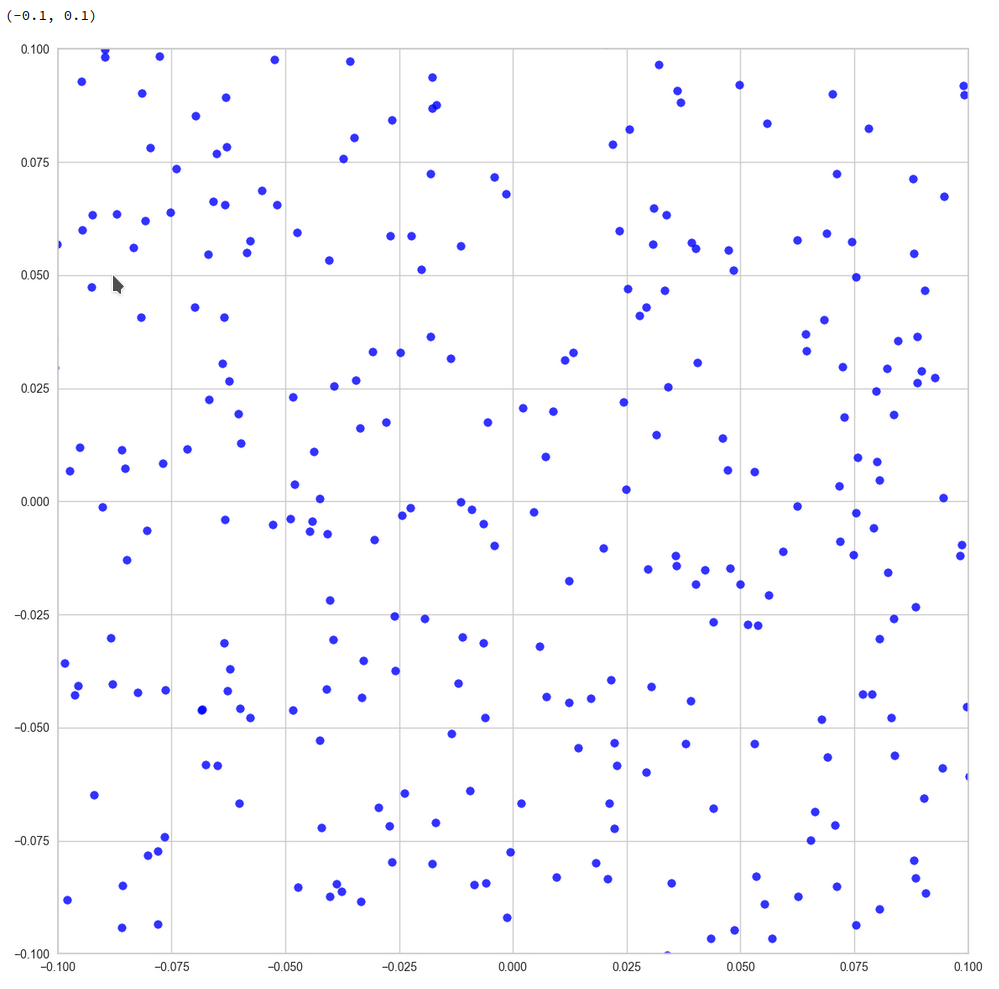Or the distribution resulting for the plane formed by the 8th and the 35th PCA axis: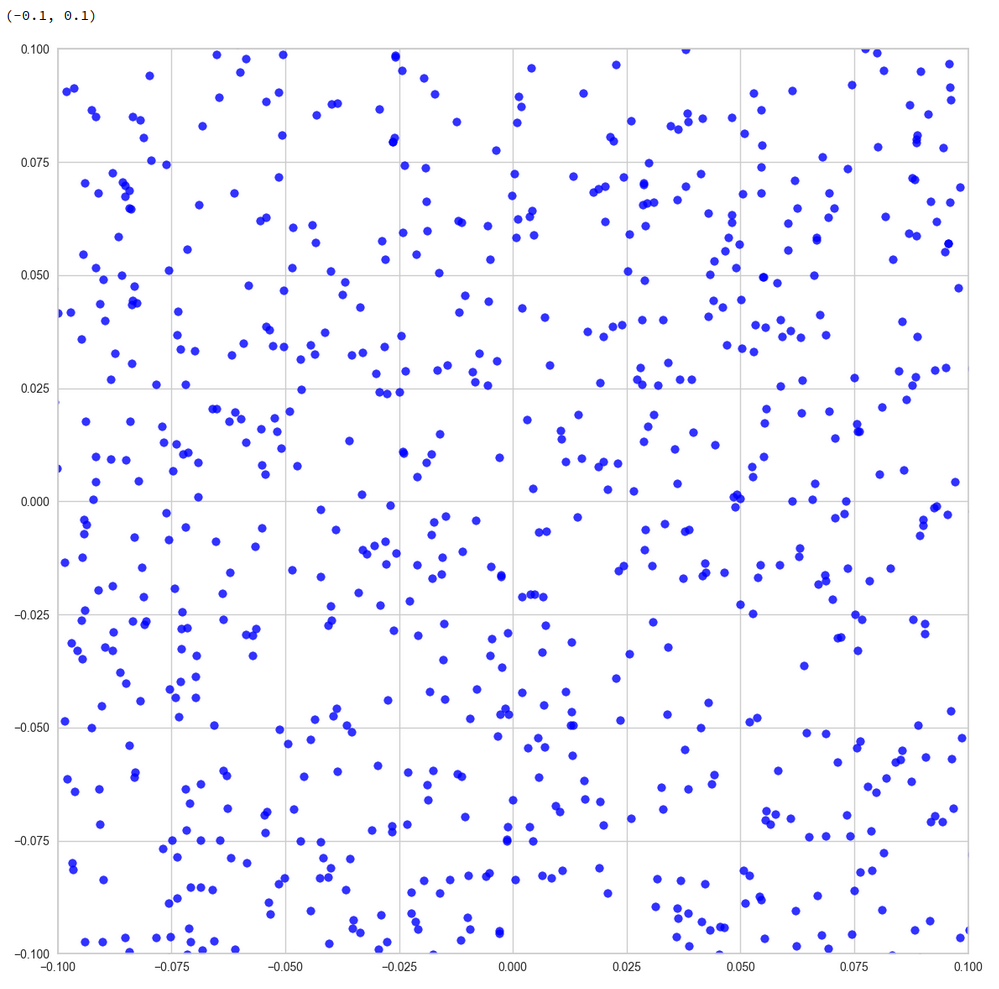We could look at other flat planes, but we do not get rid of he line like structures around void like areas. This is really a strong indication of filamental structures.

Interpretation of the line patterns:
The interesting thing is that we get lines for z-point projections onto multiple planes. What does this tell us about the structure of the filaments? In principle we have the two possibilities already named above: 1) Thin multidimensional manifolds or 2) thin and basically one-dimensional manifolds. If you think a bit about it, you will see that projections of multidimensional manifolds would not give us lines or curves on all projection planes. However curved string- or tube-like manifolds do appear as lines or line segments after a projection onto almost all flat planes. The prerequisite is that the extension of the string in other directions than its main one must really be small. The filament has to have a small diameter in all but one directions.

So, if the filaments really are one-dimensional string-like objects: Should we not see something similar in the original z-space? Let us for example look at the plane formed by axis 52 and axis 221 in the original z-space (without PCA transformation). You remember that these were axes where the distribution got elongated and had centers at -2 and 5, respectively. And indeed:

Again we see lines and voids. And this strengthens our idea about filaments as more or less one-dimensional manifolds.

The “meaningful” z-points for our CelebA data obviously get positioned on long, very thin and basically one-dimensional filaments which surround voids. And the voids are relatively large regarding their area/volume. (Reminds me of the galaxy distribution in simulations of the development of the early universe, by the way.)

Therefore: Whenever you chose a randomly positioned z-point the chance that you end up in an unpopulated region of the z-space or in a void and not on a filament is extremely big.

# Conclusion

We have used a whole set of methods to analyze the z-point distribution of an AE trained on CelebA images. We found the the z-point distribution is dominated by the number density variation along a few coordinate axes. Elongated shapes in certain directions of the latent space are very plausible on larger scales.

We found that the number density distributions along most of the coordinate axes have a thin Gaussian form with a peak at the origin and a half-with of 1. We have no real explanation for this finding. But it may be related to the fact the some dominant features of human faces show Gaussian distributions around a mean value. With Gaussians given we could however explain why the number density vs. radius showed a peak close to R=16.

A PCA analysis finds primary directions in the multidimensional space and transforms the z-point distribution into a corresponding one for orthogonal primary components axes. For logical reason we can safely assume that the corresponding projections of the z-point distribution on the new axes would still reveal existing thin filamental structures. Actually, we found lines surrounding voids independently onto which flat plane we projected the data. This finding indicates thin, elongated and curved but basically one-dimensional filaments (like curved strings or tubes). We could see the same pattern of line-like structure in projections onto flat coordinate planes in the original latent space. The volume of the void areas is obviously much bigger than the volume occupied by the filaments.

Non-linear t-SNE projections onto a 2-dim flat hyperplanes which in addition reproduce and normalize correlations on multiple scales should make things a bit fuzzier, but still show empty regions between denser areas. Our t-SNE projections all showed signs of complex correlation patterns of the z-points with a lot of empty space between curved structures.

The experiments all in all indicate that “meaningful” z-points of the training data, for which we get good reconstructions, lie within thin filaments on characteristic small length scales. The areas/volumes of the voids between the filaments instead are relatively big. This explains why chances that randomly chosen points in the z-space falls into a void are very high.

The results of the last post are consistent with the interpretation that z-points in the voids do not lead to reconstructions by the Decoder which exhibit standard objects of the training images. in the case of CelebA such z-points do not produce images with clear face or head like patterns. Face like features obviously correspond to very special correlations of z-point coordinates in the latent space. These correlations correspond to thin manifolds consuming only a tiny fraction of the z-space with a volume close zero.

# Variational Autoencoder with Tensorflow 2.8 – XIII – Does a VAE with tiny KL-loss behave like an AE? And if so, why?

This post continues my series on Variational Autoencoders [VAE] with some considerations regarding a VAE whose settings allow only for a tiny amount of the so called Kullback-Leibler [KL] loss.

So far, most of the posts in this series have covered a variety of methods (provided by Tensorflow and Keras) to control the KL loss. One of the previous posts (XI) provided (indirect) evidence that also GradientTape()-based methods for KL-loss calculation work as expected. In stark contrast to a standard Autoencoder [AE] our VAE trained on CelebA data proved its ability to reconstruct humanly interpretable images from random z-points (or z-vectors) in the latent space. Provided that the z-points lie within a reasonable distance to the origin.

We could leave it at that. One of the basic motivations to work with VAEs is to use the latent space “creatively”. This requires that the data points coming from similar training images should fill the latent space densely and without gaps between clusters or filaments. We have obviously achieved this objective. Now we could start to do funny things like to combine reconstruction with vector arithmetic in the latent space.

But to look a bit deeper into the latent space may give us some new insights. The central point of the KL-loss is that it induces a statistical element into the training of AEs. As a consequence a VAE fills the so called “latent space” in a different way than a simple AE. The z-point distribution gets confined and areas around z-points for meaningful training images are forced to get broader and overlap. So two questions want an answer:

• Can we get more direct evidence of what the KL-loss does to the data distribution in latent space?
• Can we get some direct evidence supporting the assumption that most of the latent space of an AE is empty or only sparsely populated? in contrast to a VAE’s latent space?

Therefore, I thought it would be funny to compare the data organization in latent space caused by an AE with that of a VAE. But to get there we need some solid starting point. If you consider a bit where you yourself would start with an AE vs. VAE comparison you will probably come across the following additional and also interesting questions:

• Can one safely assume that a VAE with only a very tiny amount of KL-loss reproduces the same z-point distribution vs. radius which an AE would give us?
• In general: Can we really expect a VAE with a very tiny Kullback-Leibler loss to behave as a corresponding AE with the same structure of convolutional layers?

The answers to all these questions are the topics of this post and a forthcoming one. To get some answers I will compare a VAE with a very small KL-loss contribution with a similar AE. Both network types will consist of equivalent convolutional layers and will be trained on the CelebA dataset. We shall look at the resulting data point density distribution vs. radius, clustering properties and the ability to create images from statistical z-points.

This will give us a solid base to proceed to larger and more natural values of the KL-loss in further posts. I got some new insights along this path and hope the presented data will be interesting for the reader, too.

Below and in following posts I will sometimes call the target space of the Encoder also the “z-space“.

# CelebA data to fill the latent vector-space

The training of an AE or a VAE occurs in a self-supervised manner. A VAe or an AE learns to create a point, a z-point, in the latent space for each of the training objects (e.g. CelebA images). In such a way that the Decoder can reconstruct an object (image) very close to the original from the z-point’s coordinate data. We will use the “CelebA” dataset to study the KL-impact on the z-point distribution.CelebA is more challenging for a VAE than MNIST. And the latent space requires a substantially higher number of dimensions than in the MNIST case for reasonable reconstructions. This makes things even more interesting.

The latent z-space filled by a trained AE or VAE is a multi-dimensional vector space. Meaning: Each z-point can be described by a vector defining a position in z-space. A vector in turn is defined by concrete values for as many vector components as the z-space has dimensions.

Of course, we would like to see some direct data visualizing the impact of the KL-loss on the z-point distribution which the Encoder creates for our training data. As we deal with a multidimensional vector space we cannot plot the data distribution. We have to simplify and somehow get rid of the many dimensions. A simple solution is to look at the data point distribution in latent space with respect to the distance of these points from the origin. Thereby we transform the problem into a one-dimensional one.

More precisely: I want to analyze the change in numbers of z-points within “radius“-intervals. Of course, a “radius” has to be defined in a multidimensional vector space as the z-space. But this can easily be achieved via an Euclidean L2-norm. As we expect the KL loss to have a confining effect on the z-point distribution it should reduce the average radius of the z-points. We shal later see that this is indeed the case.

Another simple method to reduce dimensions is to look at just one coordinate axis and the data distribution for the calculated values in this direction of the vector space. Therefore, I will also check the variation in the number of data points along each coordinate axis in one of the next posts.

A look at clustering via projections to a plane may be helpful, too.

# The expected similarity of a VAE with tiny KL-loss to an AE is not really obvious

Regarding the answers to the 3rd and 4th questions posed above your intuition tells you: Yes, you probably can bet on a similarity between a VAE with tiny KL-loss and an AE.

But when you look closer at the network architectures you may get a bit nervous. Why should a VAE network that has many more degrees of freedom than an AE not use both of its layers for “mu” and “logvar” to find a different distribution solution? A solution related to another minimum of the loss hyperplane in the weight configuration space? Especially as this weight-related space is significantly bigger than that of a corresponding AE with the same convolutional layers?

The whole point has to do with the following facts: In an AE’s Encoder the last flattening layer after the Conv2D-layers is connected to just one output layer. In a VAE, instead, the flattening layer feeds data into two consecutive layers (for mu and logvar) across twice as many connections (with twice as many weight parameters to optimize).

In the last post of this series we dealt with this point from the perspective of VRAM consumption. Now, its the question in how far a VAE will be similar to an AE for a tiny KL-loss.

Why should the z-points found be determined only by mu-values and not also by logvar-values? And why should the mu values reproduce the same distribution as an AE? At least the architecture does not guarantee this by any obvious means …

Well, let us look at some data.

# Structure of an AE for CelebA and its total loss after some epochs

Our test AE contains the same simple sequence of four Conv2D layers per Encoder and four 4 Conv2DTranspose layers as our VAE. See the AE’s Encoder layer structure below.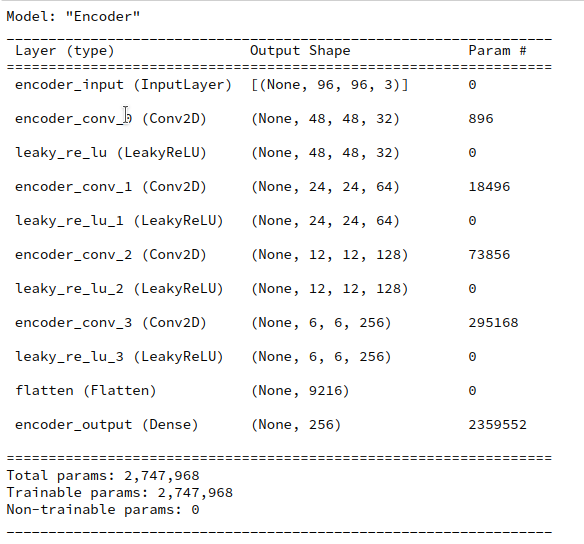A difference, however, will be that I will not use any BatchNormalizer layers in the AE. But as a correctly implemented BatchNormalization should not affect the representational powers of a VAE network for very principle reasons this should not influence the comparison of the final z-point distribution in a significant way.

I performed an AE training run for 170,000 CelebA training images over 24 epochs. The latent space has a dimension if z_dim=256. (This relatively low number of dimensions will make it easier for a VAE to confine z_points around the origin; see the discussion in previous posts).

The resulting total loss of our AE became ca. 0.49 per pixel. This translates into a total value of

AE total loss on Celeb A after 24 epochs (for a step size of 0.0005): 4515

This value results from a summation over all geometric pixels of our CelebA images which were downsized to 96×96 px (see post IX). The given value can be compared to results measured by our GradientTape()-based VAE-model which delivers integrated values and not averages per pixel.

This value is significantly smaller than values we would get for the total loss of a VAE with a reasonably big KL-loss of contribution in the order of some percent of the reconstruction loss. A VAE produces values around 4800 up to 5000. Apparently, an AE’s Decoder reconstructs originals much better than a VAE with a significant KL-loss contribution to the total loss.

But what about a VAE with a very small KL-loss? You will get the answer in a minute.

# Where does a standard Autoencoder [AE] place the z-points for CelebA data?

We can not directly plot a data point distribution in a 256-dimensional vector-space. But we can look at the data point density variation with a calculated distance from the origin of the latent space.

The distance R from the origin to the z-point for each image can be measured in terms of a L2 (= Euclidean) norm of the latent vector space. Afterward it is easy to determine the number of images within all radius intervals with e.g. a length of 0.5 e.g. between radii R

0  <  R  <  35 .

We perform the following steps to get respective numbers. We let the Encoder of our trained AE predict the z-points of all 170,000 training data

```z_points  = AE.encoder.predict(data_flow)
```

data_flow was created by a Keras DataImageGenerator to send batches of training data to the GPU (see the previous posts).

Radius values are then calculated as

```print("NUM_Images_Train = ", NUM_IMAGES_TRAIN)
for i in range(0, NUM_IMAGES_TRAIN):
sq = np.square(z_points[i])
sqrt_sum_sq = math.sqrt(sq.sum())
```

The numbers vs. radius relation then results from:

```li_rad      = []
int_width = 0.5
for i in range(0,70):
low   = int_width * i
high  = int_width * (i+1)
num   = np.count_nonzero( (ay_rad_z >= low) & (ay_rad_z < high ) )
```

The resulting curve is shown below: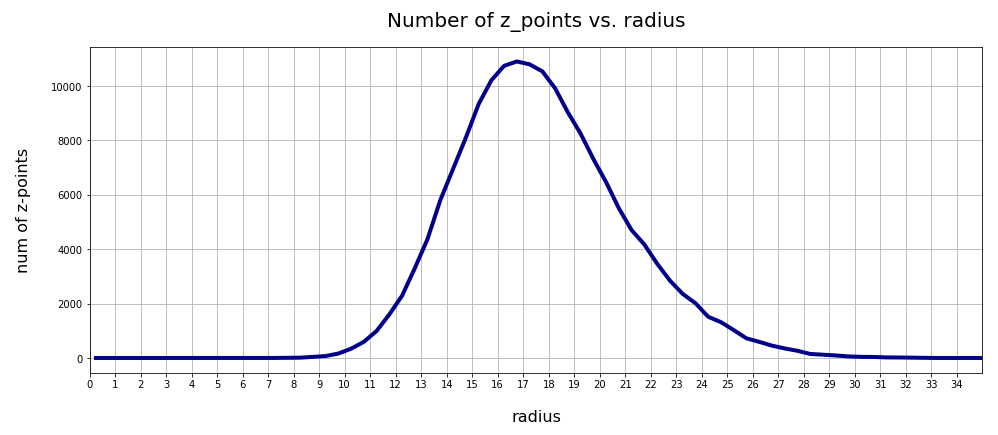There seems to be a peak around R = 16.75. So, yet another question arises:

>What is so special about the radius values of 16 or 17 ?

We shall return to this point in the next post. For now we take this result as god-given.

# Clustering of CelebA z-point data in the AE’s latent space?

Another interesting question is: Do we get some clustering in the latent space? Will there be a difference between an AE and a VAE?

A standard method to find an indication of clustering is to look for an elbow in the so called “inertia” curve for different assumed numbers of clusters. Below you find an inertia plot retrieved from the z-point data with the help of MiniBatchKMeans.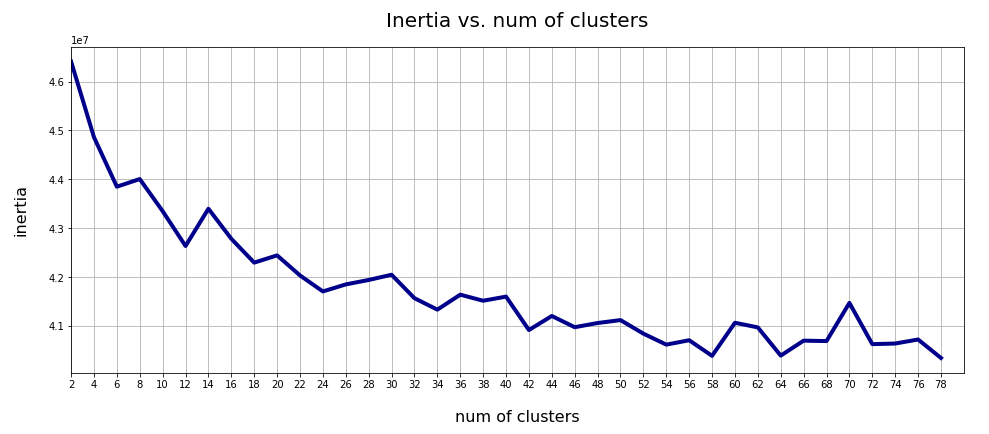This result was achieved for data taken at every second value of the number of clusters “num_clus” between 2 ≤ num_clus ≤ 80. Unfortunately, the result does not show a pronounced elbow. Instead the variation at some special cluster numbers is relatively high. But, if we absolutely wanted to define a value then something between 38 and 42 appears to be reasonable. Up to that point the decline in inertia is relatively smooth. But do not let you get misguided – the data depend on statistics and initial cluster values. When you change to a different calculation you may get something like the following plot with more pronounced spikes: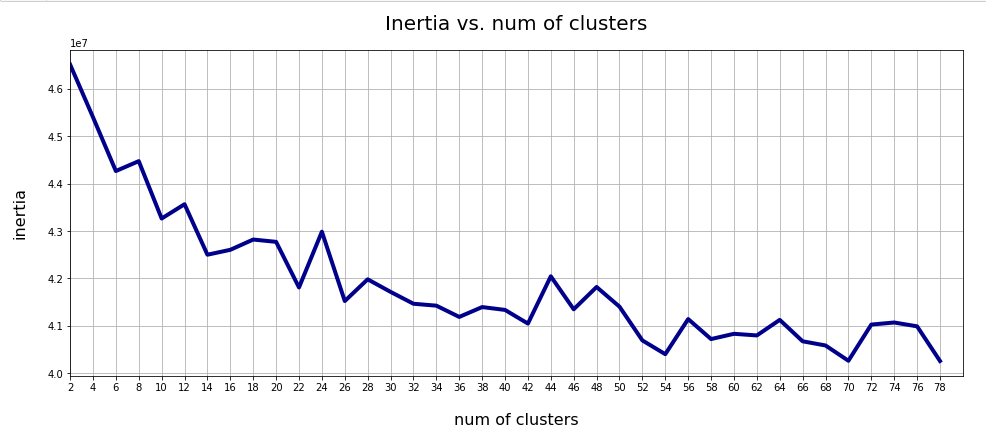This is always as sign that the clustering is not very clear – and that the clusters do not have a significant distance, at least not in all coordinate directions. Filamental structures will not be honored well by KMeans.

Nevertheless: A value of 40 is reasonable as we have 40 labels coming with the CelebA data. I.e. 40 basic features in the face images are considered to be significant and were labeled by the creators of the dataset.

## t-SNE projections

We can also have a look at a 2-dimensional t-SNE-projection of the z-point distribution. The plots below have been produced with different settings for early exaggeration and perplexity parameters. The first plot resulted from standard parameter values for sklearn’s t-SNE variant.

```tsne = TSNE(n_components=2, early_exaggeration=12, perplexity=30, n_iter=1000)
```

Other plots were produced by the following setting:

```tsne = TSNE(n_components=2, early_exaggeration=16, perplexity=10, n_iter=1000)
```

Below you find some plots of a t-SNE-analysis for different numbers and different adjusted parameters for the resulting scatter plot. The number of statistically chosen z-point varies between 20,000 and 140,000.

Number of statistical z-points: 20,000 (non-standard t-SNE-parameters)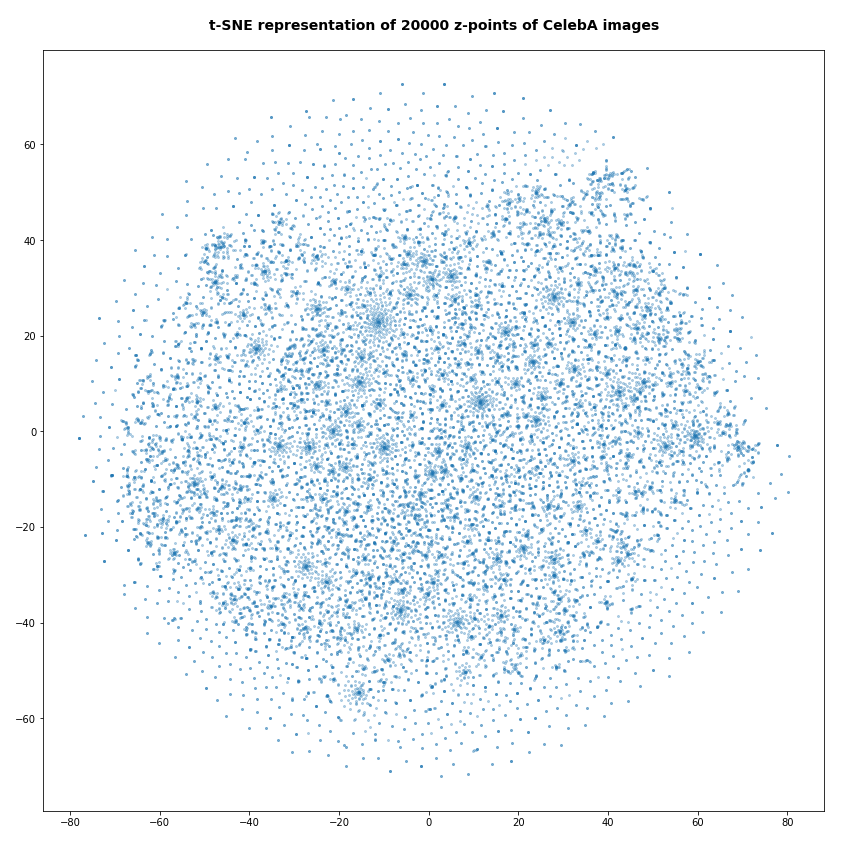Actually we see some indication of clustering, though it is not very pronounced. The clusters in the projection are not separated by clear and broad gaps. Of course a 2-dimensional projection can not completely visualize the original separations in a 256-dim space. However, we get the impression that clusters are located rather close to each other. Remember: We already know that almost all points are locates in a multidimensional sphere shell between 12 < R < 24. And more than 50% between 14 ≤ R ≤ 19.

However, how the actual distribution of meaningful z-points (in the sense of a recognizable face reconstruction) really looks like cannot be deduced from the above t-SNE analysis. The concentration of the z-points may still be one which follows thin and maybe curved filaments in some directions of the multidimensional latent space on relatively small or various scales. We shall get a much clearer picture of the fragmentation of the z-point distribution in an AE’s latent space in the next post of this series.

Number of statistical z-points: 80,000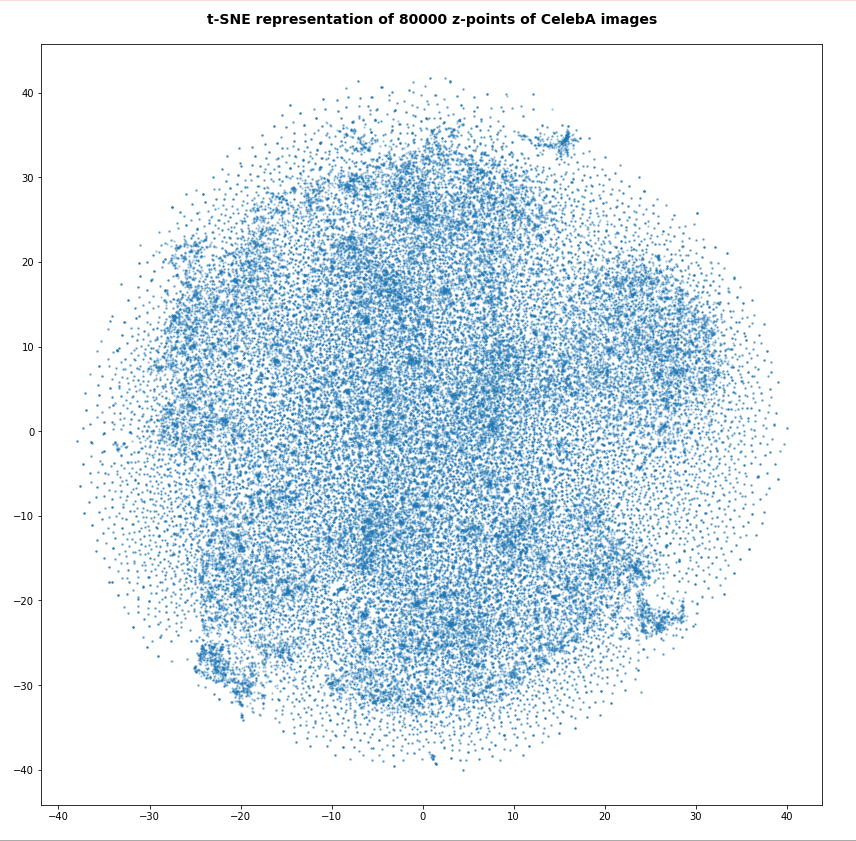For the higher number of selected z-points the room between some concentration centers appears to be filled in the projection. But remember: This may only be due to projection effects in the presently chosen coordinate system. Another calculation with the above non-standard data for perplexity and early_exaggeration gives us: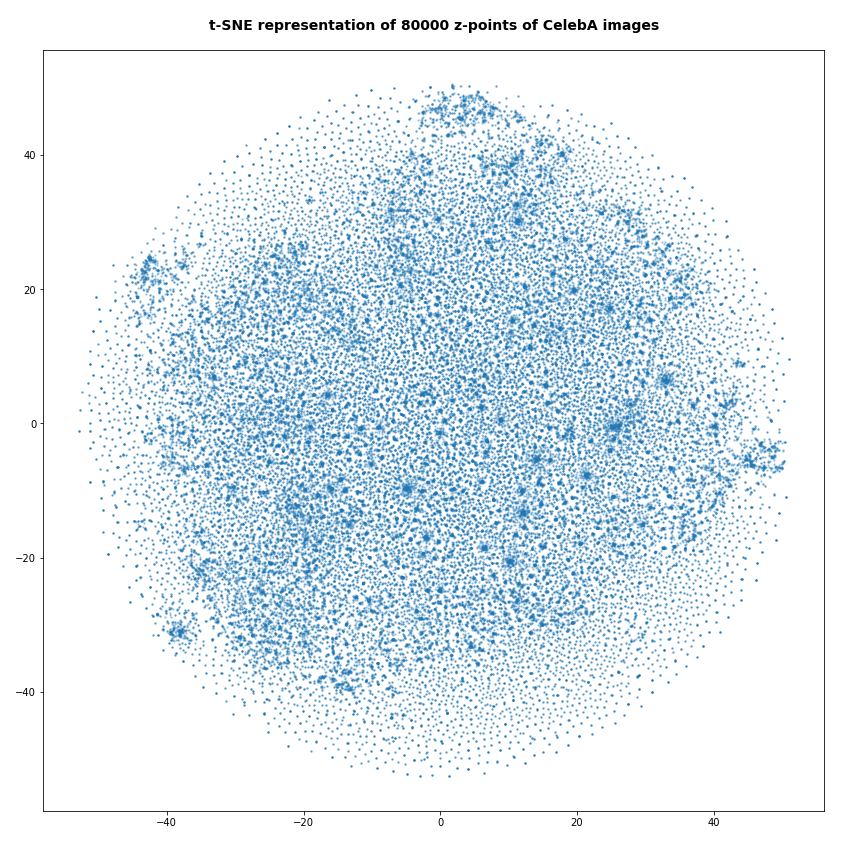Number of statistical z-points: 140,000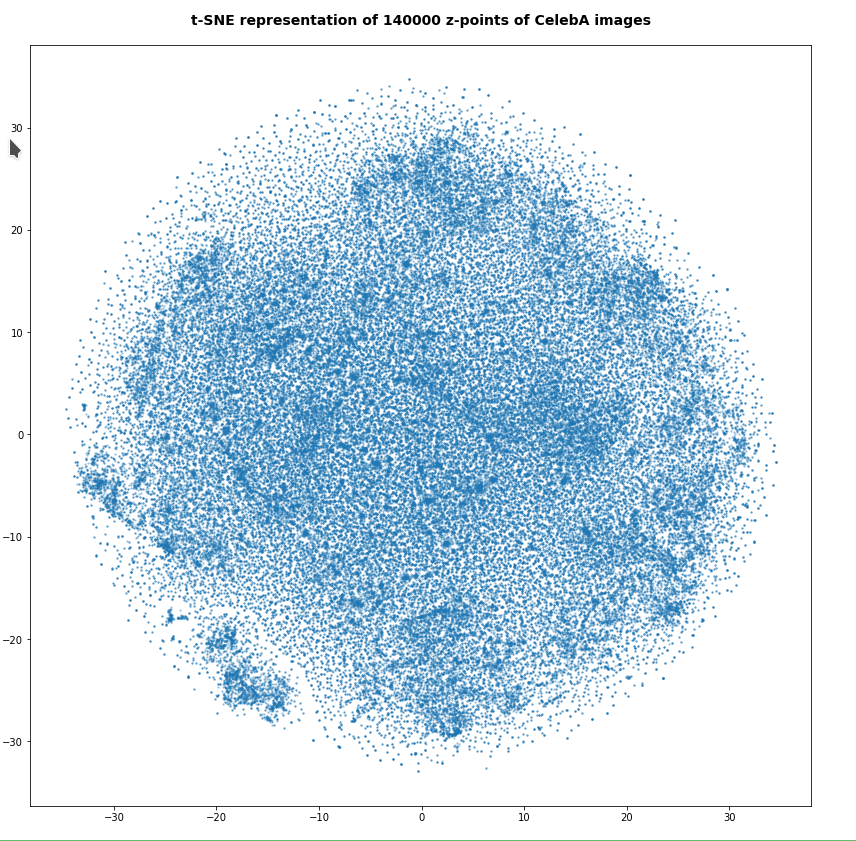Note that some islands appear. Obviously, there is at least some clustering going on. However, due to projection effects we cannot deduce much for the real structure of the point distribution between possible clusters. Even the clustering itself could appear due to overlapping two or more broader filaments along a projection line.

Whether correlations would get more pronounced and therefore could also be better handled by t-SNE in a rotated coordinate system based on a PCA-analysis remains to be seen. The next post will give an answer.

At least we have got a clear impression about the radial distribution of the z-points. And thereby gathered some data which we can compare to corresponding results of a VAE.

# Total loss of a VAE with a tiny KL-loss for CelebA data

Our test VAE is parameterized to create only a very small KL-loss contribution to the total loss. With the Python classes we have developed in the course of this post series we can control the ratio between the KL-loss and a standard reconstruction loss as e.g. BCE (binary-crossentropy) by a parameter “fact“.

For BCE

```fact = 1.0e-5
```

is a very small value. For a working VAE we would normally choose something like fact=5 (see post XI).

A value like 1.0e-5 ensures a KL loss around 0.0178 compared to a reconstruction loss of 4550, which gives us a ratio below 4.e-6. Now, what is a VAE going to do, when the KL-loss is so small?

For the total loss the last epochs produced the following values:

AE total loss on Celeb A after 24 epochs for a step size of 0.0005: 4,553

Output of the last 6 of 24 epochs.

```Epoch 1/6
1329/1329 [==============================] - 120s 90ms/step - total_loss: 4557.1694 - reco_loss: 4557.1523 - kl_loss: 0.0179
Epoch 2/6
1329/1329 [==============================] - 120s 90ms/step - total_loss: 4556.9111 - reco_loss: 4556.8940 - kl_loss: 0.0179
Epoch 3/6
1329/1329 [==============================] - 120s 90ms/step - total_loss: 4556.6626 - reco_loss: 4556.6450 - kl_loss: 0.0179
Epoch 4/6
1329/1329 [==============================] - 120s 90ms/step - total_loss: 4556.3862 - reco_loss: 4556.3682 - kl_loss: 0.0179
Epoch 5/6
1329/1329 [==============================] - 120s 90ms/step - total_loss: 4555.9595 - reco_loss: 4555.9395 - kl_loss: 0.0179
Epoch 6/6
1329/1329 [==============================] - 118s 89ms/step - total_loss: 4555.6641 - reco_loss: 4555.6426 - kl_loss: 0.0178

```

This is not too far away from the value of our AE. Other training runs confirmed this result. On four different runs the total loss value came to lie between

VAE total loss on Celeb A after 24 epochs: 4553 ≤ loss ≤ 4555 .

# VAE with tiny KL-loss – z-point density distribution vs. radius

Below you find the plot for the variation of the number density of z-points vs. radius for our VAE: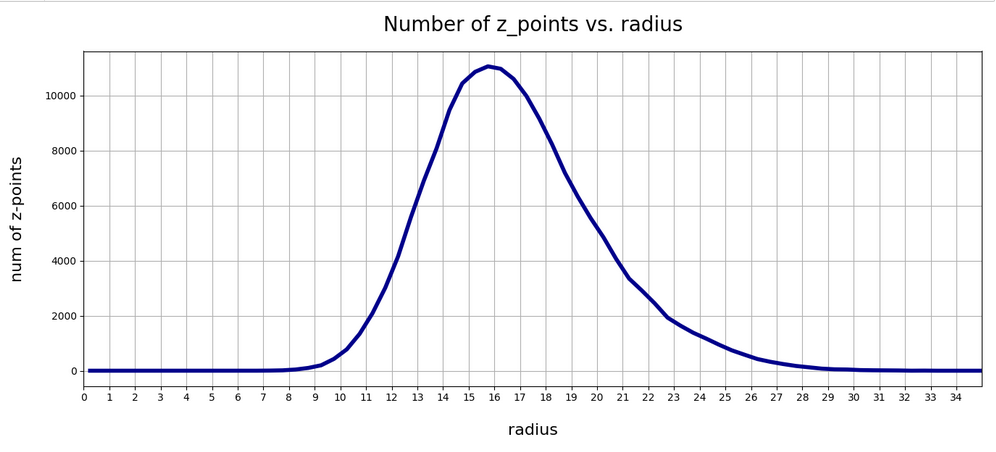Again, we get a maximum close to R = rad = 16. The maximum value lies a bit below the one found for a KL-loss-free AE. But all in all the form and width of the distribution of the VAE are very comparable to that of our test AE.

Can this result be reproduced?
Unfortunately not at a 100% of test runs performed. There are two main reasons:

1. Firstly, we can not be sure that a second minimum does not exist for a distribution of points at bigger radii. This may be the case both for the AE and the VAE!
2. Secondly, we have a major factor of statistical fluctuation in our game:
The epsilon value which scales the logvar-contribution to the loss in the sampling layer of the Encoder may in very seldom cases abruptly jump to an unreasonable high value. A Gaussian covers extreme values, although the chances to produce such a value are pretty small. and a Gaussian is invilved in the calculation of z-points by our VAE.

Remember that the z-point coordinates are calculated via the the mu and logvar tensors according to

```
z = mu + B.exp(log_var / 2.) * epsilon
```

See Variational Autoencoder with Tensorflow 2.8 – VIII – TF 2 GradientTape(), KL loss and metrics for respective code elements of the Encoder.

So, a lot depends on epsilon which is calculated as a statistically fluctuating quantity, namely as

```epsilon = B.random_normal(shape=B.shape(mu), mean=0., stddev=1.)
```

Is there a chance that the training process may sometimes drive the system to another corner of the weight-loss configuration space due to abrupt fluctuations? With the result for the z-point distribution vs. radius that it may significantly deviate from a distribution around R = 16? I think: Yes, this is possible!

From some other training runs I actually have an indication that there is a second minimum of the cost hyperplane with similar properties for higher average radius-values, namely for a distribution with an average radius at R ≈ 19.75. I got there after changing the initialization of the weights a bit.

Another indication that the cost surface has a relative rough structure and that extreme fluctuations of epsilon and a resulting gradient-fluctuation can drive the position of the network in the weight configuration space to some strange corners. The weight values there can result in different z-point distributions at higher average radii. This actually happened during yet another training run: At epoch 22 the Adam optimizer suddenly directed the whole system to weight values resulting in a maximum of the density distribution at R = 66 ! This appeared as totally crazy. At the same time the KL-loss also jumped to a much higher value.

When I afterward repeated the run from epoch 18 this did not happen again. Therefore, a statistical fluctuation must have been the reason for the described event. Such an erratic behavior can only be explained by sudden and extreme changes of z-point data enforcing a substantial change in size and direction of the loss gradient. And epsilon is a plausible candidate for this!

So far I had nothing in our Python classes which would limit the statistical variation of epsilon. The effects seen spoke for a code change such that we do not allow for extreme epsilon-values. I set limits in the respective part of the code for the sampling layer and its lambda function

```        # The following function will be used by an eventual Lambda layer of the Encoder
def z_point_sampling(args):
'''
A point in the latent space is calculated statistically
around an optimized mu for each sample
'''
mu, log_var = args # Note: These are 1D tensors !
epsilon = B.random_normal(shape=B.shape(mu), mean=0., stddev=1.)
if abs(epsilon) >= 5:
epsilon *= 5. / abs(epsilon)
return mu + B.exp(log_var / 2.) * epsilon * self.enc_v_eps_factor
```

This stabilized everything. But even without these limitations on average three out of 4 runs which I performed for the VAE ran into a cost minimum which was associated with a pronounced maximum of the z-point-distribution around R ≈ 16. Below you see the plot for the fourth run: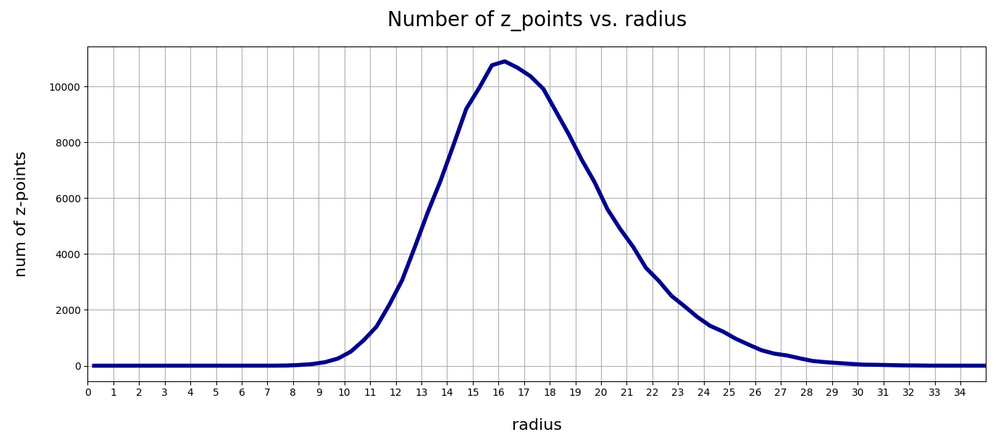So, there is some chance that the degrees of freedom associated with the logvar-layer and the statistical variation for epsilon may drive a VAE into other local minima or weight parameter ranges which do not lead to a z-point distribution around R = 16. But after the limitation of epsilon fluctuations all training runs found a loss minimum similar to the one of our simple AE – in the sense that it creates a z-point density distribution around R ≈ 16.

# VAE with tiny KL-loss: Inertia and clustering of the CelebA data?

Our VAE gives the following variation of the inertia vs. the number of assumed clusters: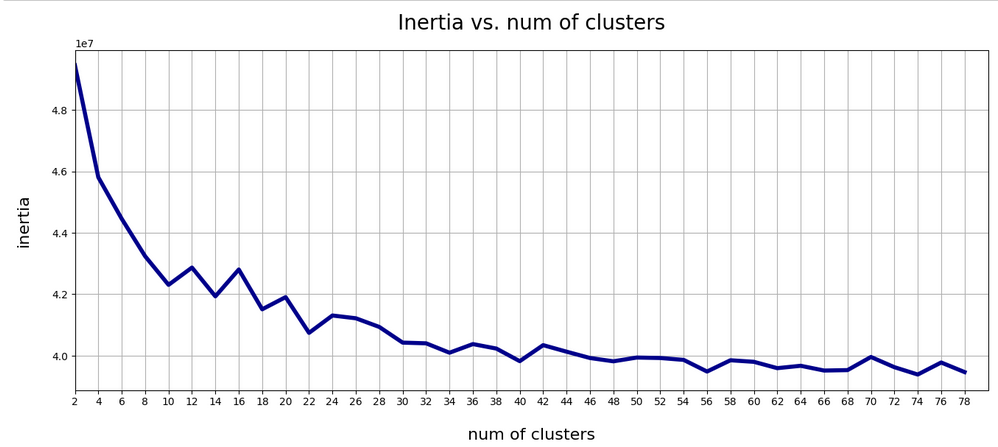This also looks pretty similar to one of the plots shown for our AE above.

# t-SNE for our VAE with a tiny KL loss

Below you find t-SNE plots for 20,000, 80,000 and 140,000 images:

Number of statistical z-points: 20,000 (non-standard t-SNE-parameters)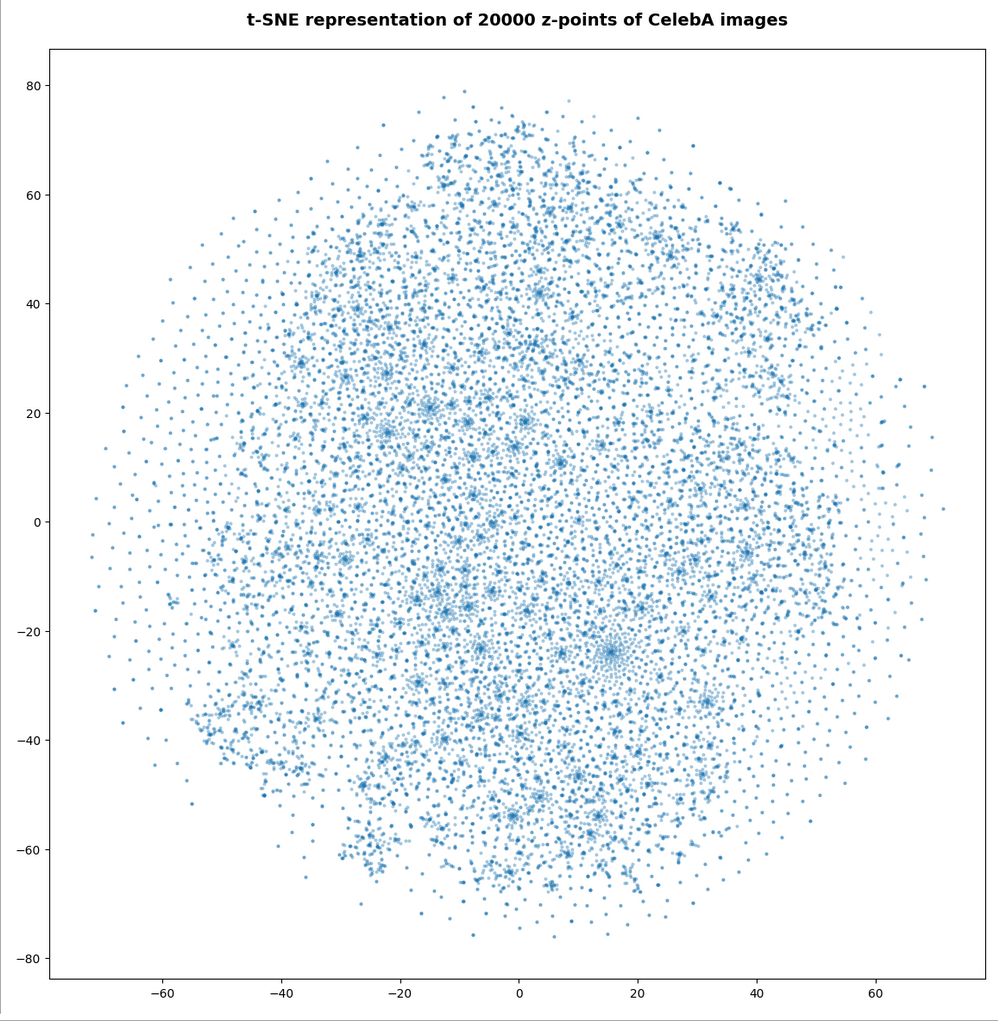This is quite similar to the related image for the AE. You just have to rotate it.

Number of statistical z-points: 80,000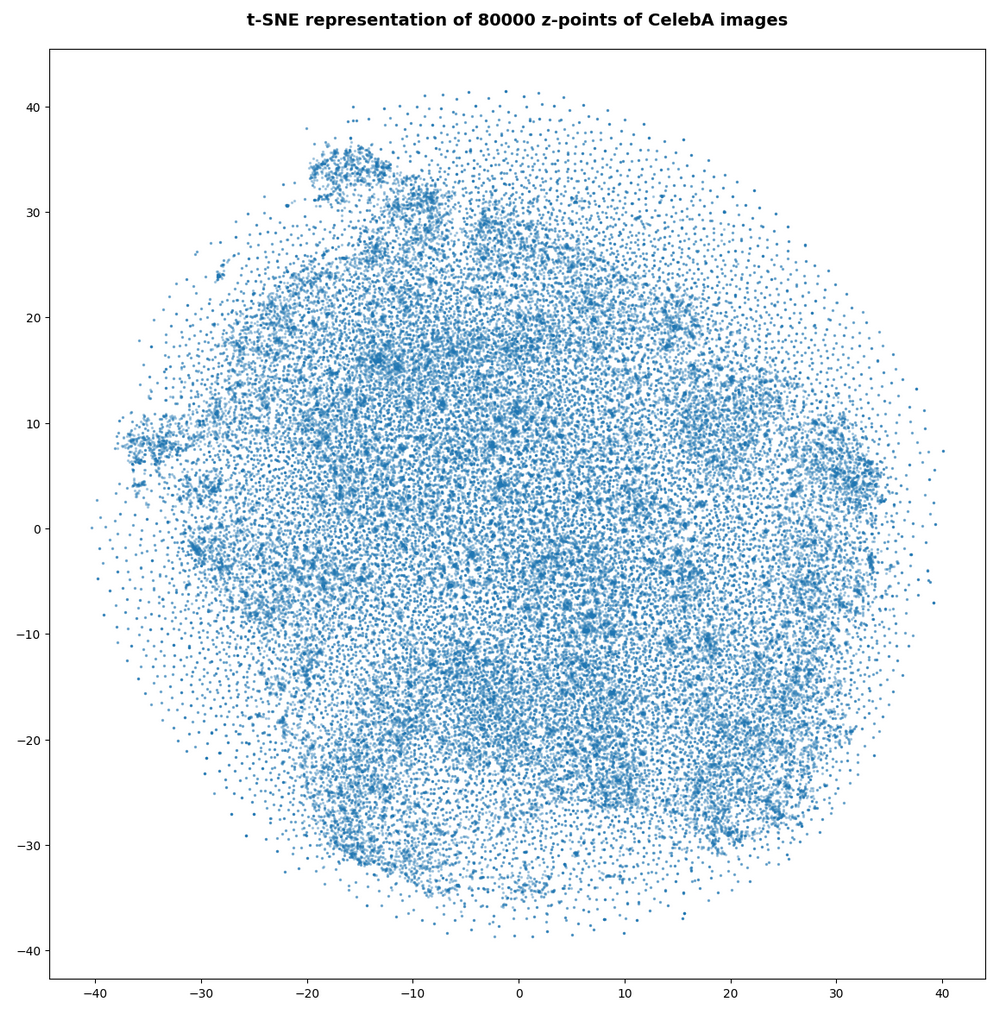Number of statistical z-points: 140,000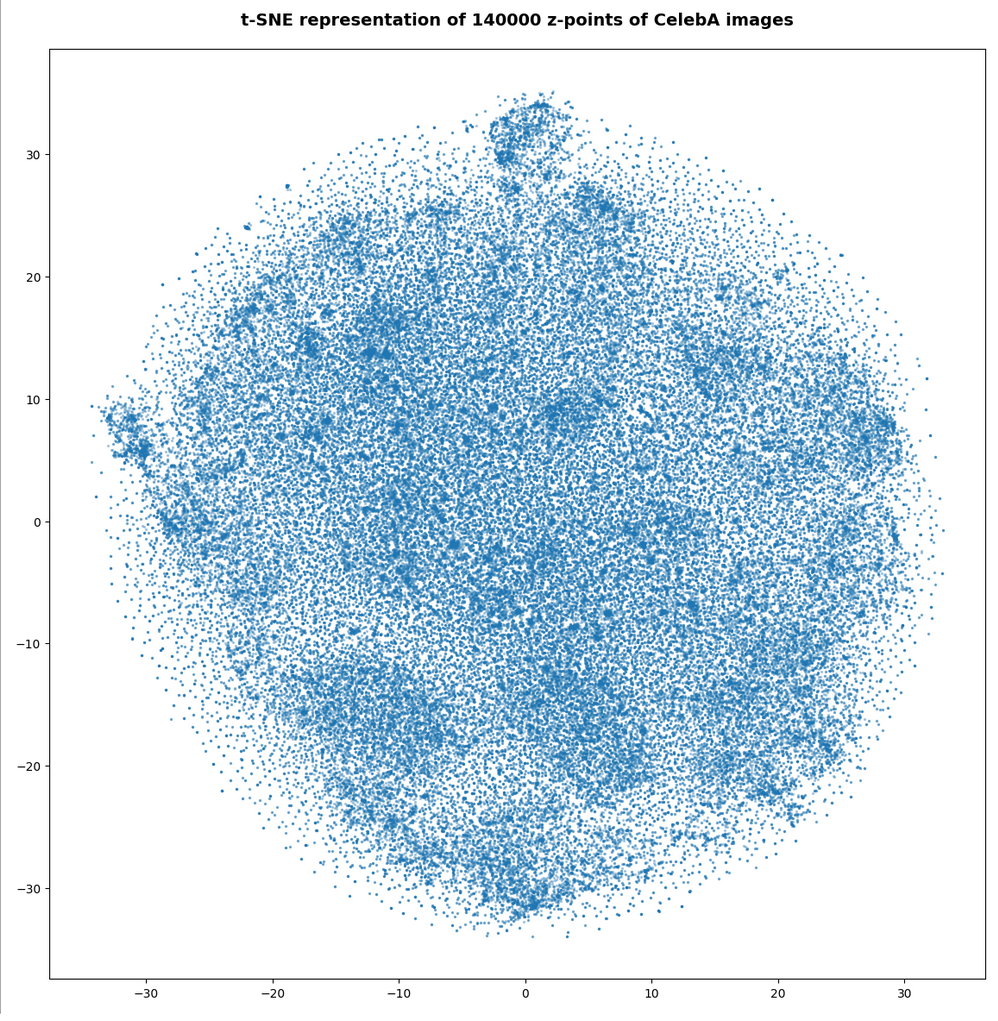All in all we get very similar indications as from our AE that some clustering is going on.

# VAE with tiny KL-loss: Should its logvar values become tiny, too?

Besides reproducing a similar z-point distribution with respect to radius values, is there another indication that a VAE behaves similar to an AE? What would be a clear sign that the similarity really exists on a deeper level of the layers and their weights?

The z-vector is calculated from the mu and logvar-vectors by:

```z = mu + exp(logvar/2)*epsilon
```

with epsilon coming from a normal distribution. Please note that we are talking about vectors of size z_dim=256 per image.

If a VAE with a tiny KL-loss really becomes similar to an AE it should define and set its z-points basically by using mu-values, only, and not by logvar-values. I.e. the VAE should become intelligent enough to ignore the degrees of freedom associated with the logvar-layer. Meaning that the z-point coordinates of a VAE with a very small Kl-loss should in the end be almost identical to the mu-component-values.

Ok, but to me it was not self-evident that a VAE during its training would learn

• to produce significant mu-related weight-values, only,
• and to keep the weight values for the connections to the logvar-layer so small that the logvar-impact on the z-space position gets negligible.

Before we speculate about reasons: Is there any evidence for a negligible logvar-contribution to the z-point coordinates or, equivalently, to the respective vector components?

# A VAE with tiny KL-loss produces tiny logvar values …

To get some quantitative data on the logvar impact the following steps are appropriate:

1. Get the size and algebraic sign of the logvar-values. Negative values logvar < -3 would be optimal.
2. Measure the deviation between the mu- and z_points vector components. There should only be a few components which show significant values &br; abs(mu – z) > 0.05
3. Compare the the radius-value determined by z-components vs. the radius values derived from mu-components, only, and measure the absolute and relative deviations. The relative deviation should be very small on average.

## Some values of logvar, (z – mu), z-radii and z-radius-deviations for a VAE with small KL-loss

Regarding the maximum value of the logvar’s vector-components I found

```3.4 ≥ max(logvar) ≥ -3.2. # for 1 up to 3 components out of a total 45.52 million components
```

The first value may appear to be big for a component. But typically there are only 2 (!) out of 170,000 x 256 = 43.52 million vector components in an interval of [-3, 5]. On the component level I found the following minimum, maximum and average-values

```Maximum value for logvar:  -2.0205276
Minimum value for logvar:  -24.660698
Average value for logvar:  -13.682616
```

The average value of logvar is pretty pleasing: Such big negative values indeed render the logvar-impact on the position of our z-points negligible. So we should only find very small deviations of the mu-components from the z-point components. And, actually, the maximum of the deviation between a z_point component and a mu component was delta_mu_z = 0.26:

```Maximum (z_points - mu) = delta_mu_z = 0.26  # on the component level
```

There were only 5 out of the 45.52 million components which showed an absolute deviation in the interval

```0.05 < abs(delta_mu_z) < 2.
```

The rest was much, much smaller!

What about radius values? Here the situation looks, of course, even better:

```max radius defined by z  :  33.10274
min radius defined by z  :  6.4961233
max radius defined by mu :  33.0972
min radius defined by mu :  6.494558

avg_z:      16.283989
avg_mu:     16.283972

max absolute difference :  0.018045425
avg absolute difference :  0.00094899256

max relative difference  :   0.00072147313
avg relative difference  :   6.1240215e-05
```

As expected, the relative deviations between z- and mu-based radius values became very small.

In another run (the one corresponding to the second density distribution curve above) I got the following values:

```Maximum value for logvar:  3.3761873
Minimum value for logvar:  -22.777826
Average value for logvar:  -13.4265175

avg_z:  17.37412
avg_mu: 17.374104

max delta rad relative :   0.012512478
avg delta rad relative :   6.5660715e-05
```

This tells us that the z-point distributions may vary a bit in their width, their precise center and average values. But, overall they appear to be similar. Especially with respect to a relative negligible contribution of logvar-terms to the z-point position. The relative impact of logvar on the radius value of a z-point is of the order 6.e-5, only.

All the above data confirm that a trained VAE with a very small KL-loss primarily uses mu-values to set the position of its z-points. During training the VAE moves along a path to an overall minimum on the loss hyperplane which leads to an area with weights that produce negligible logvar values.

# Explanation of the overall similarity of a VAE with tiny KL-loss to an AE

o far we can summarize: Under normal conditions the VAE’s behavior is pretty close to that of a similar AE. The VAE produces only small logvar values. z-point coordinates are extremely close to just the mu-coordinates.

Can we find a plausible reason for this result? Looking at the cost-hyperplane with respect to the Encoder weights helps:

The cost surface of a VAE spans across a space of many more weight parameters than a corresponding AE. The reason is that we have weights for the connection to the logvar-layer in addition to the weights for the mu-layer (or a single output layer as in a corresponding AE). But if we look at the corner of the weight-vector-space where the logvar-related values are pretty small, then we would at least find a local (if not global) loss minimum there for the same values of the mu-related weight parameters as in the corresponding AE (with mu replacing the z-output).

So our question reduces to the closely related question whether the old minimum of an AE remains at least a local one when we shift to a VAE – and this is indeed the case for the basic reason that the KL-contributions to the height of the cost-hyperplane are negligibly small everywhere (!) – even for higher logvar-related values.

This tells us that a gradient descent algorithm should indeed be able to find a cost minimum for very small values of logvar-related weights and for weight-values related to the mu-layer very close to the AE’s weight-values for direct connections to its output layer. And, of course, with all other weight parameter of the VAE-Decoder being close to the values of the weights of a corresponding AE. At least under the condition that all variable quantities really change smoothly during training.

# Does a VAE with small KL-loss produce reasonable face images?

A last test to confirm that a VAE with a very small KL-loss operates as an comparable AE is a trial to create images with recognizable human faces from randomly chosen points in z-space. Such a trial should fail! I just show you three results – one for a normal distribution of the z-point components. And two for equidistant distribution of component values up to 3, 8 and 16:

z-point coordinates from normal distribution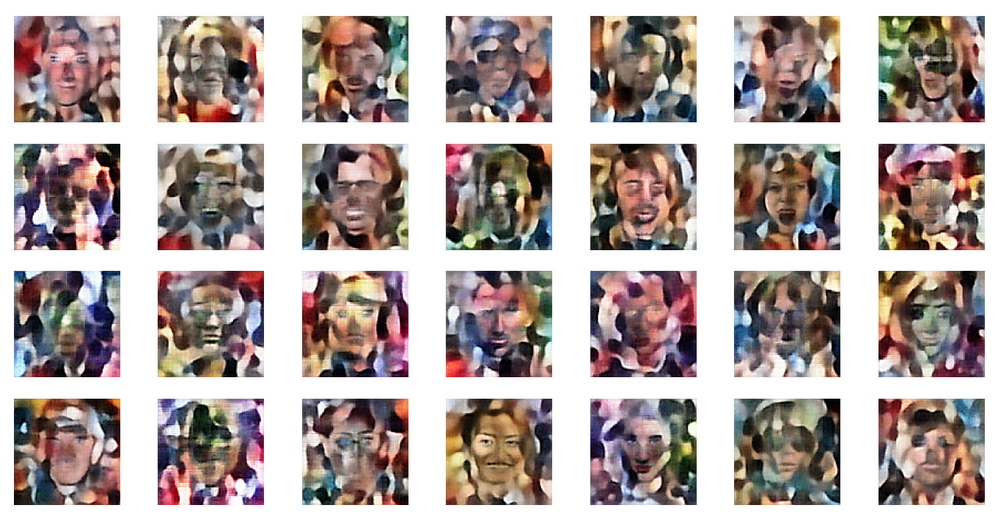z-point coordinates from equidistant distribution in [-2,2]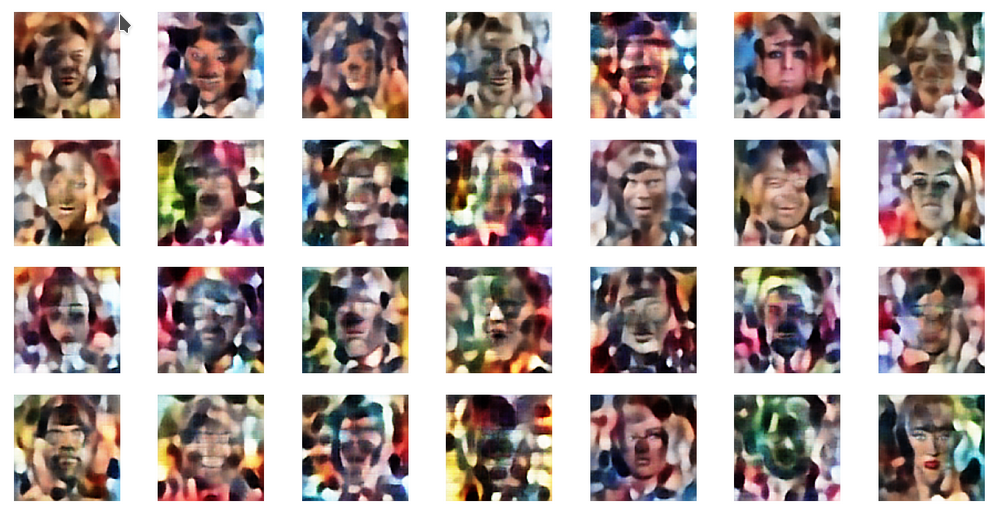z-point coordinates from equidistant distribution in [-10,10]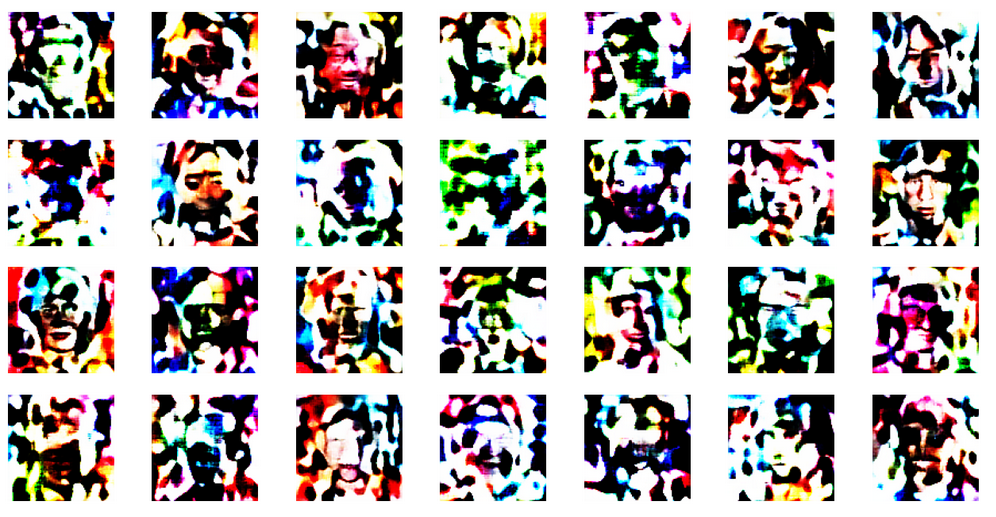This reminds us very much about the behavior of an AE. See: Autoencoders, latent space and the curse of high dimensionality – I.

The z-point distribution in latent space of a VAE with a very small KL-loss obviously is as complicated as that of an AE. Neighboring points of a z-point which leads to a good image produce chaotic images. The transition path from good z-points to other meaningful z-points is confined to a very small filament-like volume.

# Conclusion

A trained VAE with only a tiny KL-loss contribution will under normal circumstances behave similar to an AE with a the same hidden (convolutional) layers. It may, however, be necessary to limit the statistical variation of the epsilon factor in the z-point calculation based on mu– and logvar-values.

The similarity is based on very small logvar-values after training. The VAE creates a z-point distribution which shows the same dependency on the radius as an AE. We see similar indications and patterns of clustering. And the VAE fails to produce human faces from random z-points in the latent space – as a comparable AE.

We have found a plausible reason for this similarity by comparing the minimum of the loss hyperplane in the weight-loss parameter space with a corresponding minimum in the weight-loss space of the VAE – at a position with small weights for the connection to the logvar layers.

The z-point density distribution shows a maximum at a radius between 16 and 17. The z-point distribution basically has a Gaussian form. In the next post we shall look a bit closer at these findings – and their origin in Gaussian distributions along the coordinate axes of the latent space. After an application of a PCA analysis we shall furthermore see that the z-point distribution in an AE’s latent vector space is indeed fragmented and shows filaments on certain length scales. A VAE with a tiny KL-loss will show the same fragmentation.

In further forthcoming post we shall afterward investigate the confining and at the same time blurring impact of the KL-loss on the latent space. Which will make it usable for creative purposes.

And let us all who praise freedom not forget:
The worst fascist, war criminal and killer living today is the Putler. He must be isolated at all levels, be denazified and sooner than later be imprisoned. Long live a free and democratic Ukraine!

# Variational Autoencoder with Tensorflow 2.8 – XII – save some VRAM by an extra Dense layer in the Encoder

I continue with my series on Variational Autoencoders [VAEs] and related methods to control the KL-loss.

After having successfully trained a VAE with CelebA data, we have shown that our VAE can afterward create images with human-like looking faces from statistically selected data points (z-points) in its latent space. We still have to analyze the confinement of the z-point distribution due to the KL-loss a bit in more depth. But before we turn to this topic I want to briefly discuss an option to reduce the VRAM requirements of the VAE’s Encoder.

# Limited VRAM – a problem for ML training runs on older graphics cards

In my opinion exploring the field of Machine Learning on a PC should not be limited to people who can afford a state of the art graphics card with a lot of VRAM. One could use Google’s Colab – but … I do not want to go into tax and personal data politics here. I really miss an EU-wide platform that offers services like Google Colab.

Anyway, a reduction of VRAM consumption may be decisive to be able to perform training runs for CNN-based VAEs on older graphic cards . Not only concerning VRAM limits but also regarding computational time: The less VRAM the weight parameters of our VAE models require the bigger we can size the batches the GPU operates on and the more CPU time we may potentially save. At least in principle. Therefore, we should consider the amount of trainable parameters of a neural network model and reduce them if possible.

# The number of parameters depends heavily on the connections to the mu and logvar-layer of the Encoder

When you print out the layer structure and related parameters of a VAE (see below) you will find that the Encoder requires more parameters than the Decoder. Around twice as many. A closer look reveals:

It is the transition from the convolutional part of the Encoder to its Dense layers for mu and logvar which plays an important role for the number of weight parameters.

For a layer structure comprising 4 Conv2D layers, related filters=(32,64,128,256) and an input image size of (96,96,3) pixels we arrive at a Flatten-layer of 9216 neurons at the end of the convolutional part of the Encoder. For z_dim = 512 the direct connections from the Flatten-layer to both the mu- and logvar-layers lead to more the 9.4 million (float32) parameters for the Encoder. This is the absolutely dominant part of all required 9.83 million parameters of the Encoder. In contrast the Decoder part requires a total of 5.1 million parameters, only.

This is due to the fact that the flattened layer supplies input to two connected layers before output is created by yet another layer. In the Decoder, instead, only one layer, namely the input layer is connected to the flattened layer ahead of the first Conv2DTranspose layer.

In the case of z_dim=256 we arrive at around half of the parameters, i.e. 4.9 million parameters for the Encoder and around 2.76 million for the Decoder.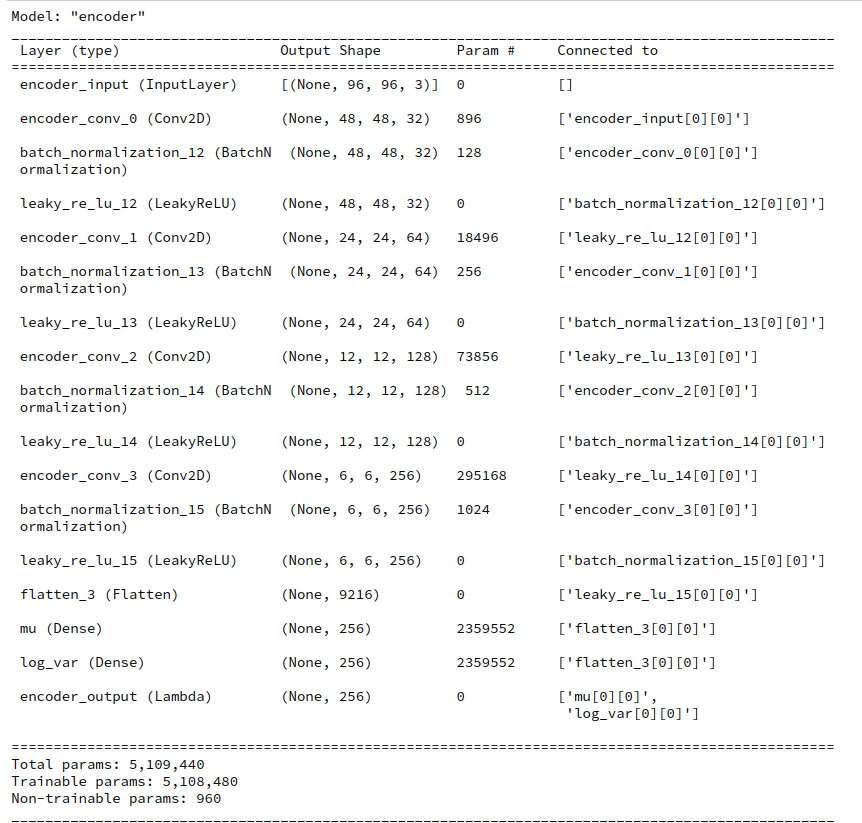It is obvious that the existence of two layers for the variational parameters inside the Encoder is the source of the high parameter number on the Encoder side.

## Would a reduction of convolutional layers help to reduce the weight parameters?

A reduction of Conv2D-layers in the Encoder would of course reduce the parameters for the weights between the convolutional layers. But turning to only three convolutional layers whilst keeping up a stride value of stride=2 for all filters would raise the already dominant number of parameters after the flattened layer by a factor of 4!

So, one has to work with a delicate balance between the number of convolutional layers and the eventual number of maps at the innermost layer and the size of these maps. They determine the number of neurons and related weights on the flattening layer:

From the perspective of a low total number of parameters you should consider higher stride values when reducing the number of Conv2D-layers.

On the other hand side using more than 4 convolutional layers would reduce the resolution of the maps of the innermost Conv2D layer below a usable threshold for reasonable mu and logvar values.

Off topic remark: All in all it seems to be reasonable also to think about ResNets of low depth instead of plain CNNs to keep weight numbers under control.

# An intermediate dense layer ahead of the mu- and logvar-layers of the Encoder?

The reader who followed the posts in this series may have looked at the recipe which F. Chollet has discussed in his Keras documentation on VAEs. See:
https://keras.io/ examples/ generative/vae/.

There is an element in Chollet’s Encoderstructure which one easily can overlook at first sight. In his example for the MNIST dataset Chollet adds an intermediate Dense layer between the Flatten-layer and the layers for mu and logvar.

```...
x = layers.Flatten()(x)
x = layers.Dense(16, activation="relu")(x)
z_mean = layers.Dense(latent_dim, name="z_mean")(x)
z_log_var = layers.Dense(latent_dim, name="z_log_var")(x)
...
```

In the special case of MNIST an intermediate layer seems appropriate for bridging the gap between an input dimension of 784 to z_dim = 2. You do not expect major problems to arise from such a measure.

But: This intermediate layer introduced by Chollet also has the advantage of reducing the total number of trainable parameters substantially.

We could try something similar for our network. But here we have to be a bit more careful as we work in a latent space of much higher dimensions, typically with z_dim >= 256. Here we are in dilemma as we want to keep the intermediate dimension relatively high for using as much information as possible coming from the maps of the last Conv2D-layers. A fair compromise seems to be to use at least the dimension of the mu and varlog-layers, namely z_dim.

For z_dim=256 an additional Dense layer of the same size would reduce the total number of Encoder parameters from 5.11 million to 2.88 million.If we took the dimension of the intermediate layer to be 384 we would still go down with the total Encoder parameters to 4.13 million. So an additional Dense layer really saves us some VRAM.

# Images constructed by a VAE model with an additional Dense layer in the Encoder

Will an additional dense layer have a negative impact on our VAE’s ability to create images from randomly chosen z-points in the latent space?

Let us try it out. To include an option for an additional Dense layer in the Encoder related part of our class “MyVariationalAutoencoder()” is a pretty simple task. I leave this to the reader. Note that if we choose the dimension of the additional Dense layer to be exactly z_dim there is no need to change the reconstruction logic and layer structure of the Decoder. Also for other choices for the size of the Dense layer I would refrain from changing the Decoder.

I used z_dim=256 for the extra layer’s size. Then I repeated the experiments described in my last post. Some results for random z-points picked from a normal distribution in all coordinates are shown below: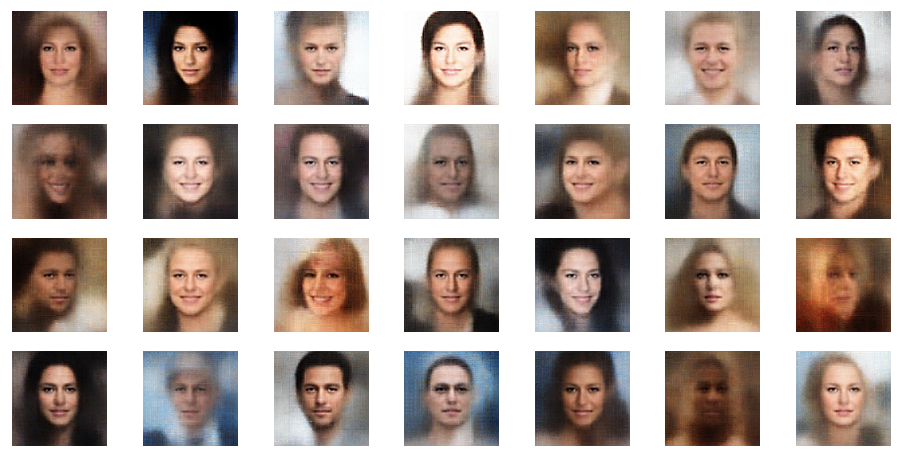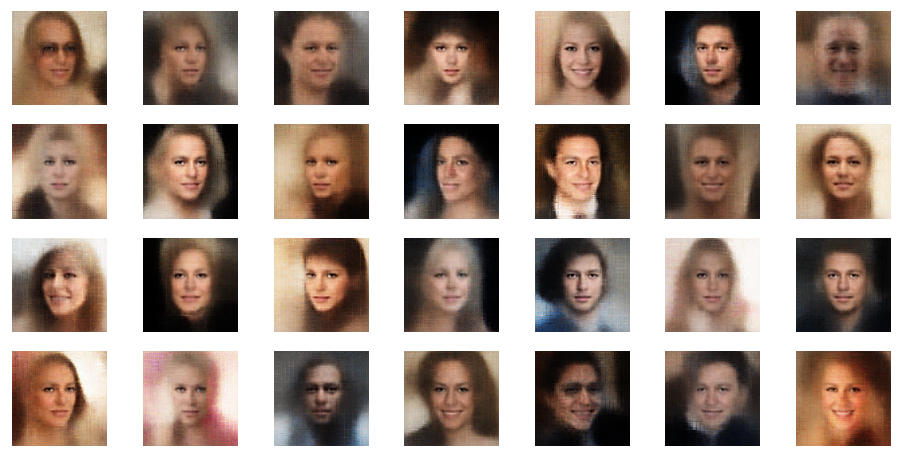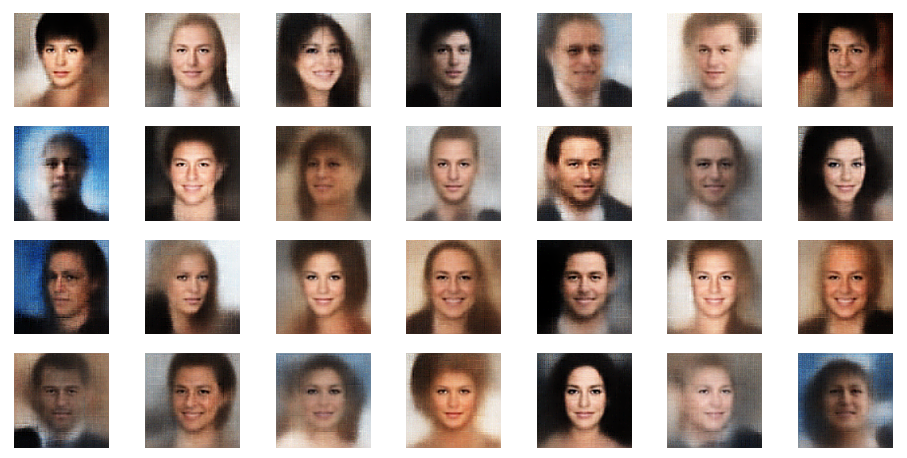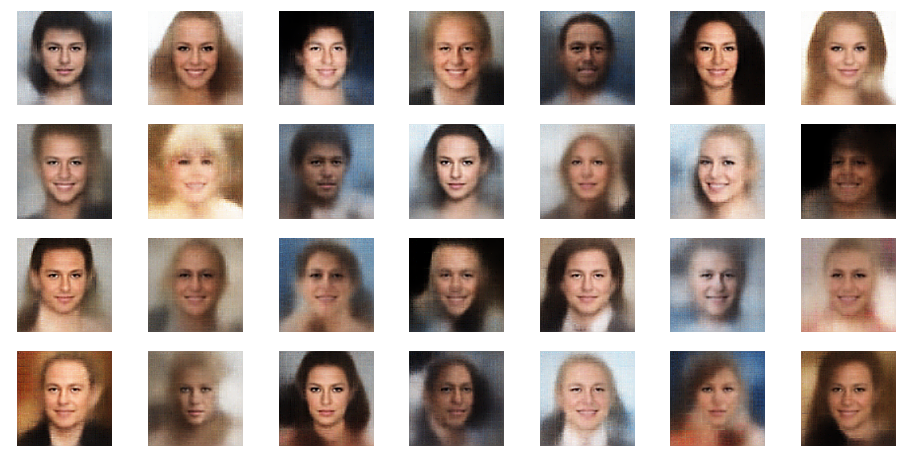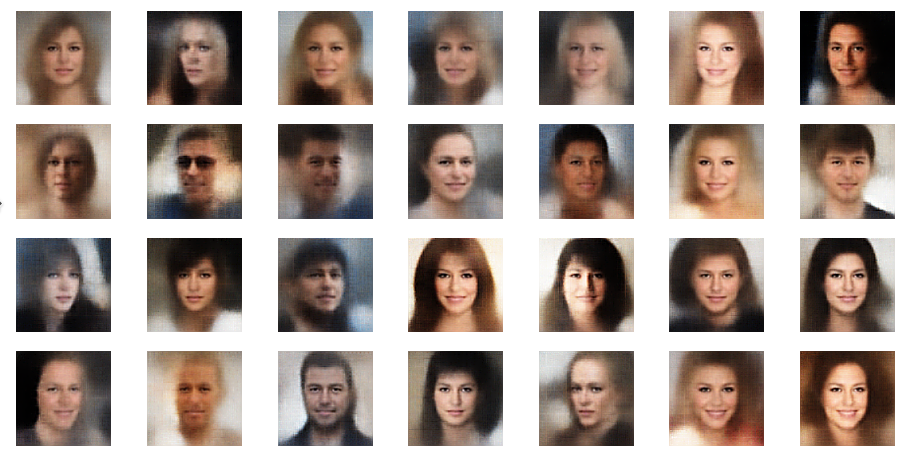So we see that from the generative point of view an extra Dense layer does not hurt too much.

# What have we gained?

First and foremost:

We found a simple method to reduce the VRAM consumption of the Encoder.

But I have to admit that this method did NOT save any GPU time during training as long as I kept the size of image batches equally big as before (128). The reason is:

Due to the extra layer more matrix operations have to be performed than before, although some of matrixes becae smaller. On my old graphics card a full epoch with 170,000 (96×96) images takes around 120 secs – with or without an extra Encoder layer. Unfortunately, increasing the size of the batches the DataImageGenerator feeds into the GPU from 128 images to 256 did not change the required GPU time very much. More tests showed that a size of 128 already gave me an optimal turnaround time per epoch on my old graphics card (960 GTX).

# Conclusion

An extra intermediate Dense layer between the Flatten-layer and the mu- and logvar-layers of the Encoder can help us to save some VRAM during the training of a VAE. Such a layer does not lead to a visible reduction of the quality of VAE-generated images from randomly selected points in the latent-space.

In the next post of this series
Variational Autoencoder with Tensorflow 2.8 – XIII – Does a VAE with tiny KL-loss behave like an AE? And if so, why?
we will compare a VAE with only a tiny contribution of KL-loss to the total loss with a corresponding AE. We shall investigate their similarity regarding their z-point distributions. This will give us a solid basis to investigate the impact of higher KL-loss values on the latent space in more detail afterwards.